# R S Aggarwal Solutions for Class 10 Maths Chapter 7 Triangles

R S Aggarwal Solutions for Class 10 Chapter 7 Triangles benefit the students in understanding concepts thoroughly. All questions are created by subject experts using step-by-step problem solving approach. Class 10 R S Aggarwal Solutions help the students to analyze their shortcomings so that they overcome and clear their doubts on concepts. Chapter 7 triangle is based on triangles and its important theorem results. Students can download free pdf and practice various problems on triangles.

## Download PDF of R S Aggarwal Solutions for Class 10 Chapter 7 Triangles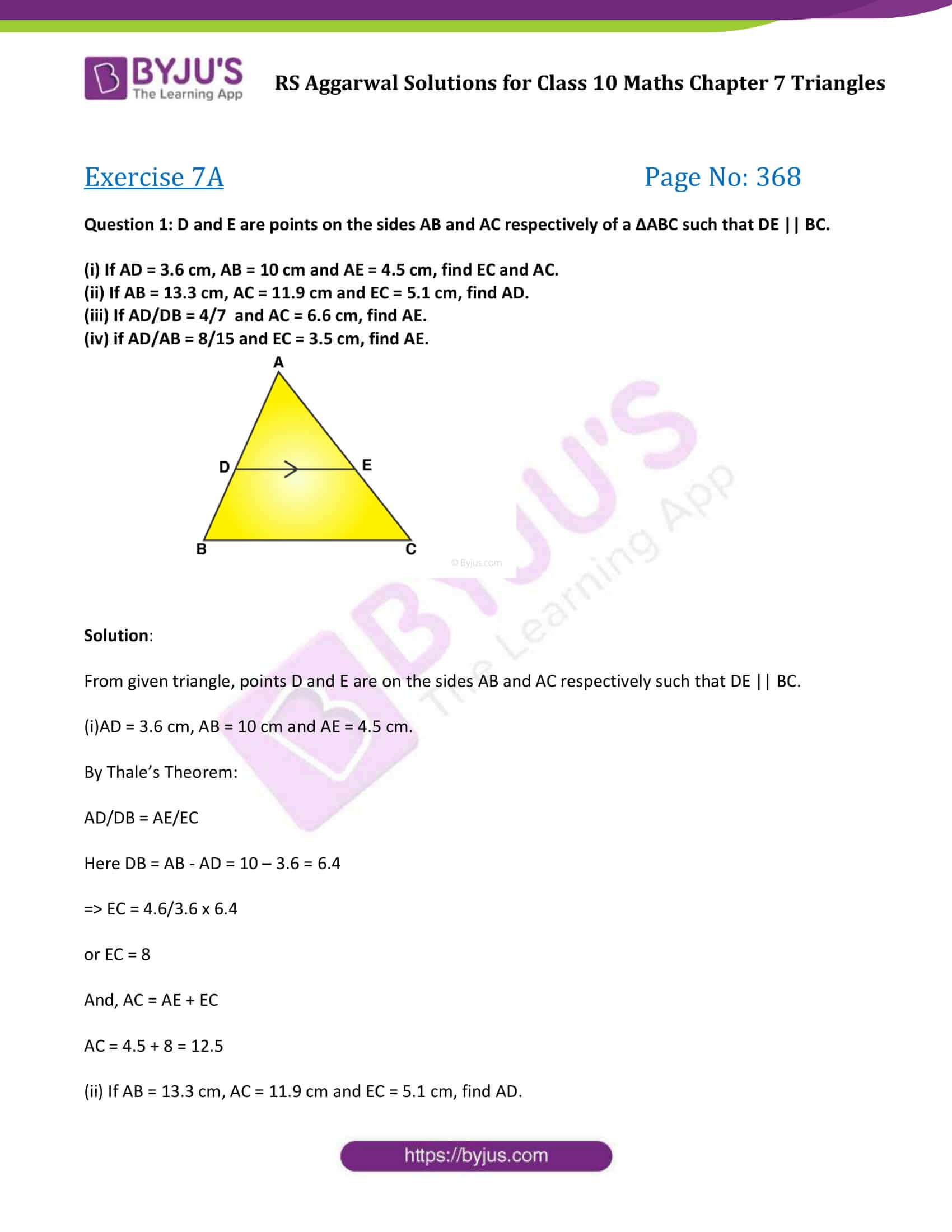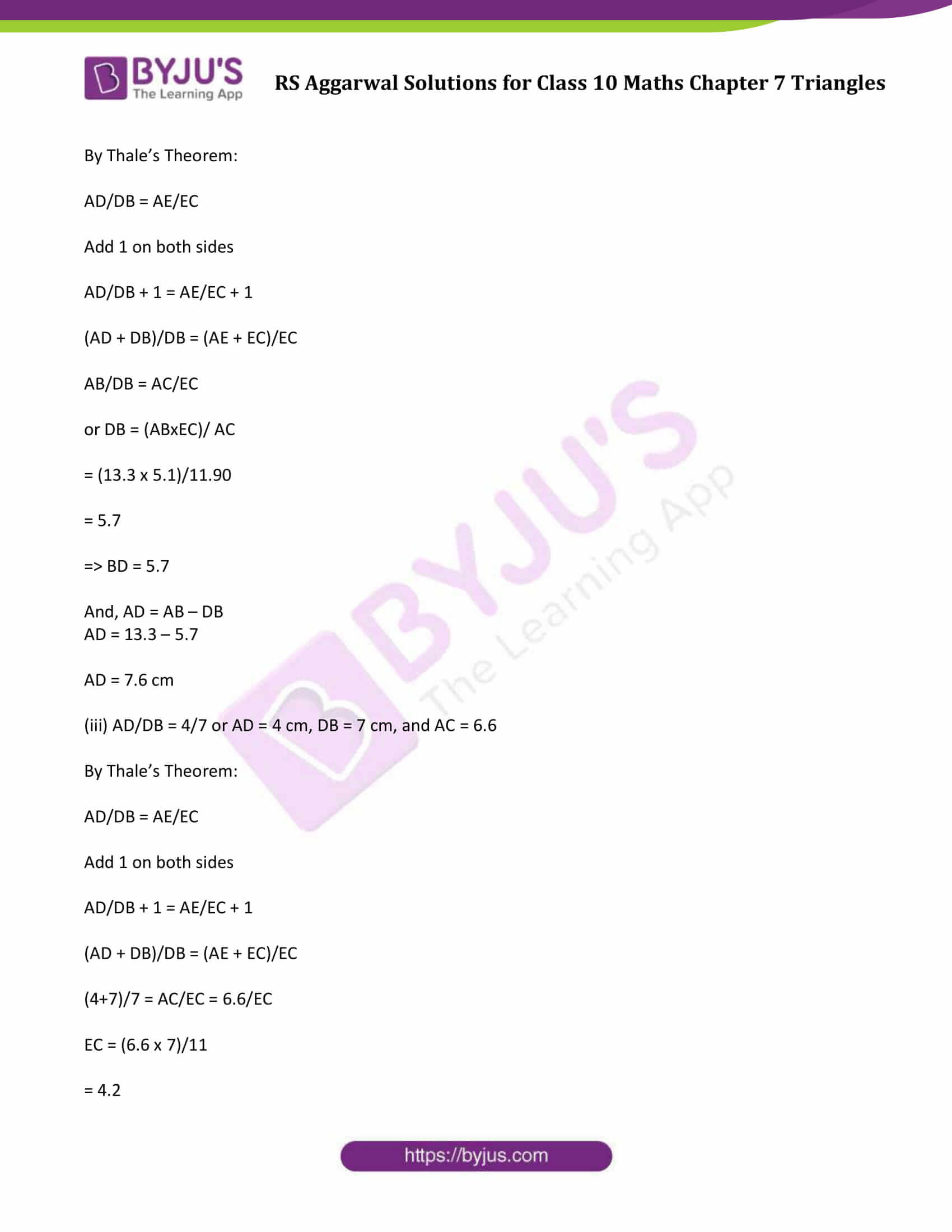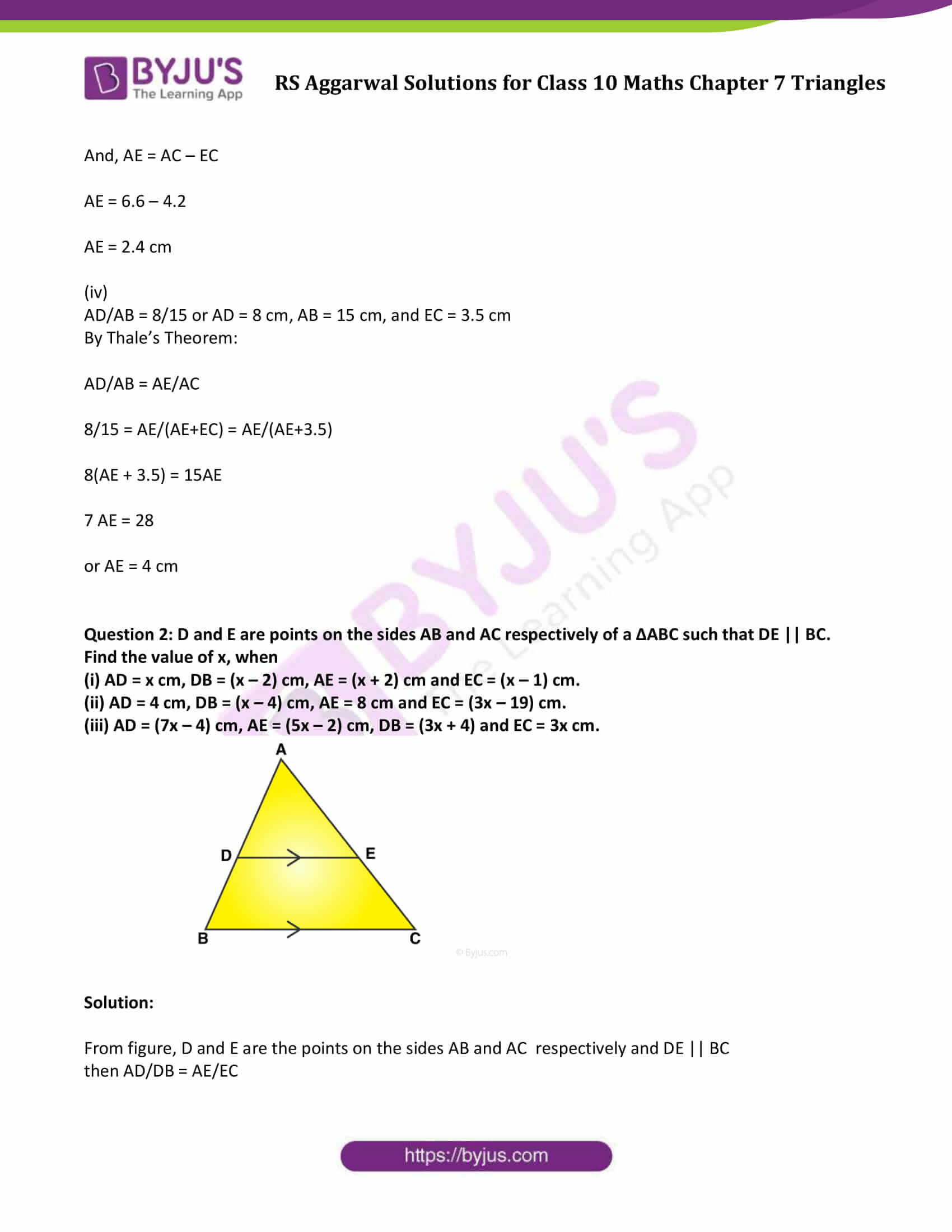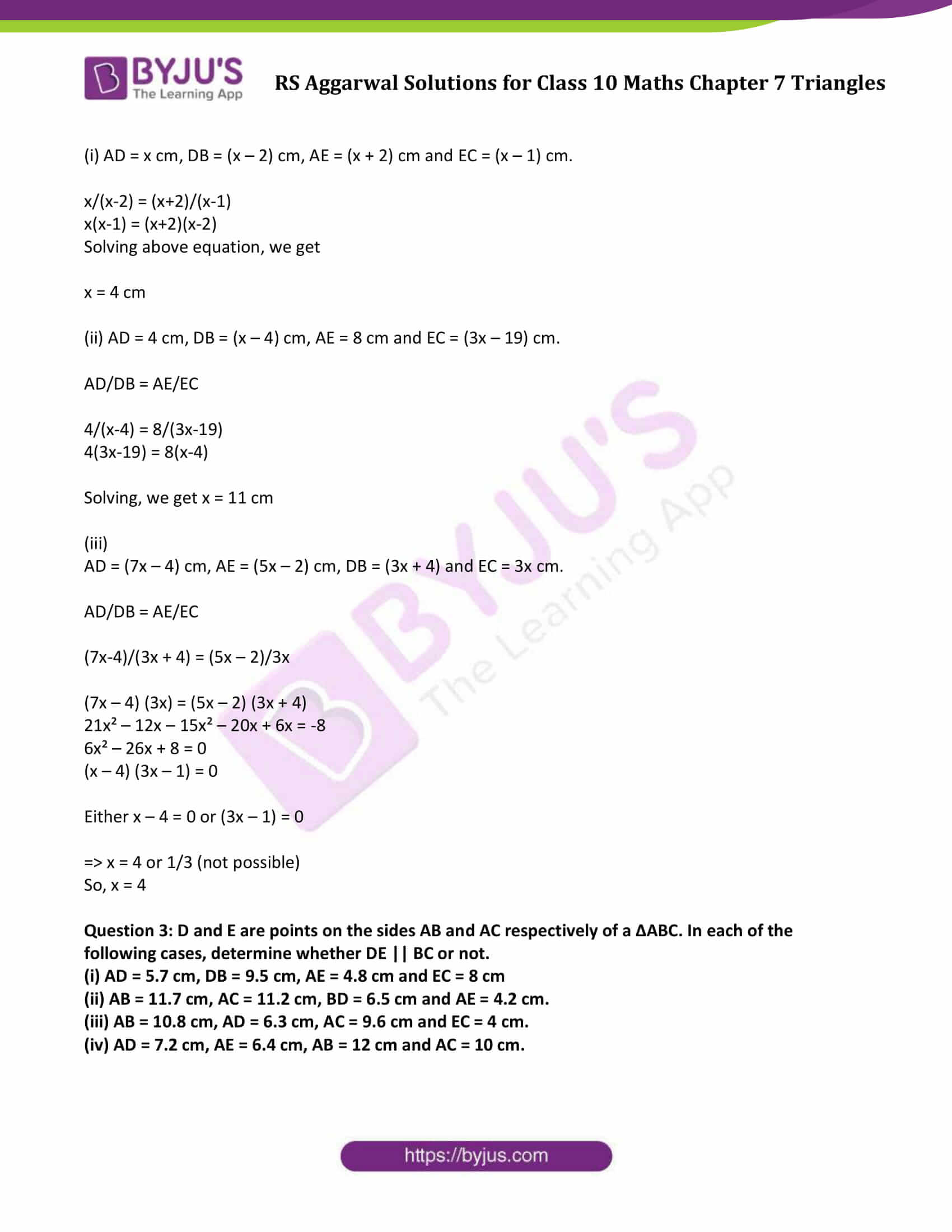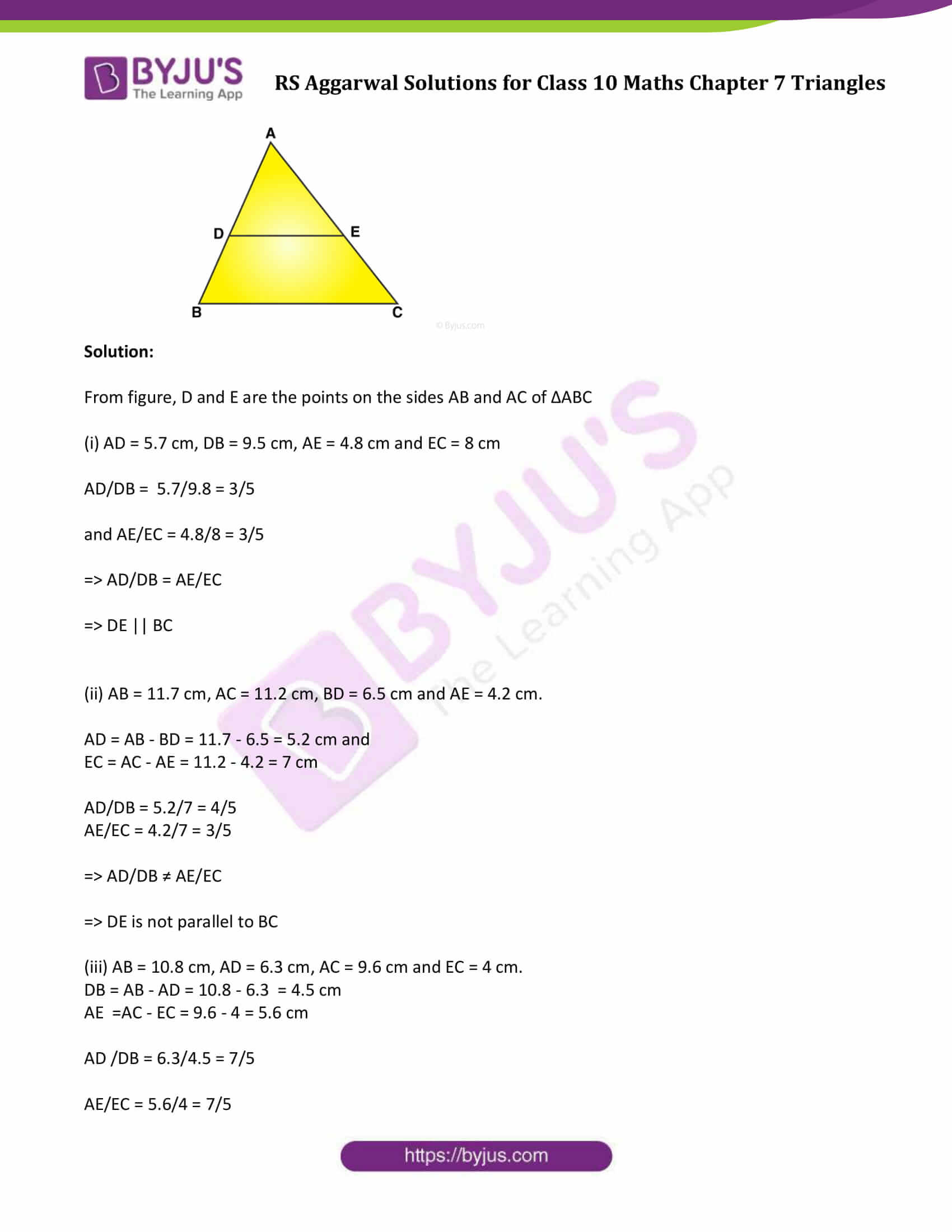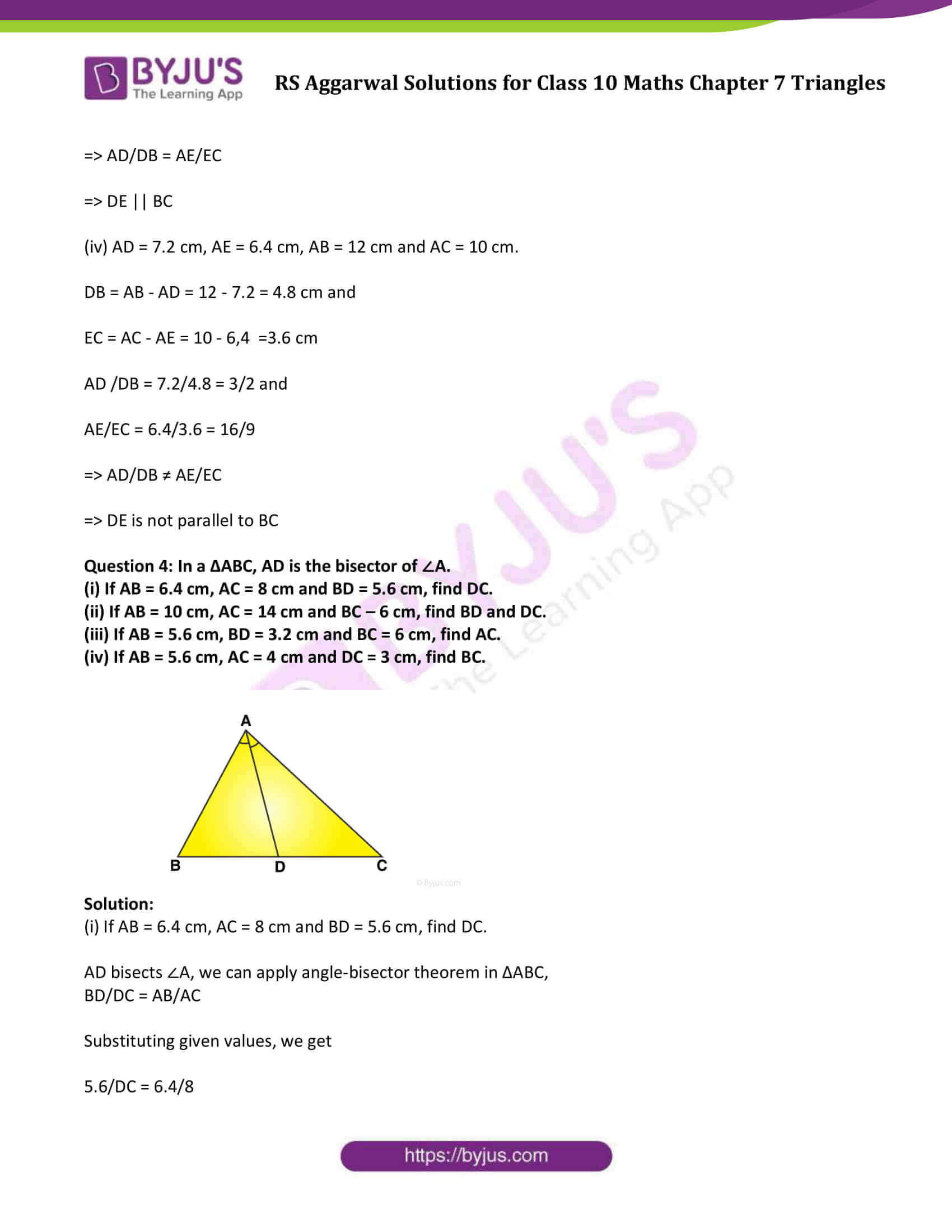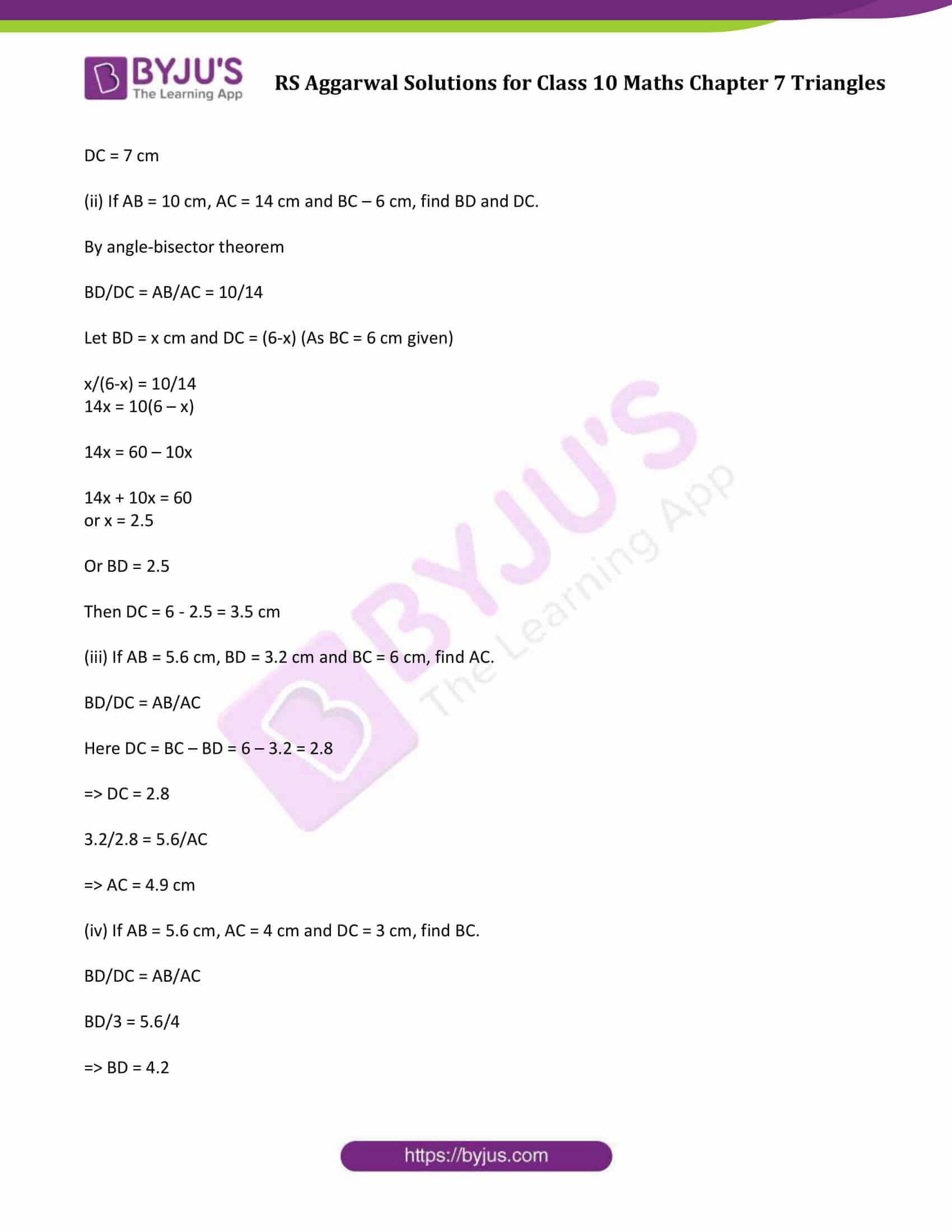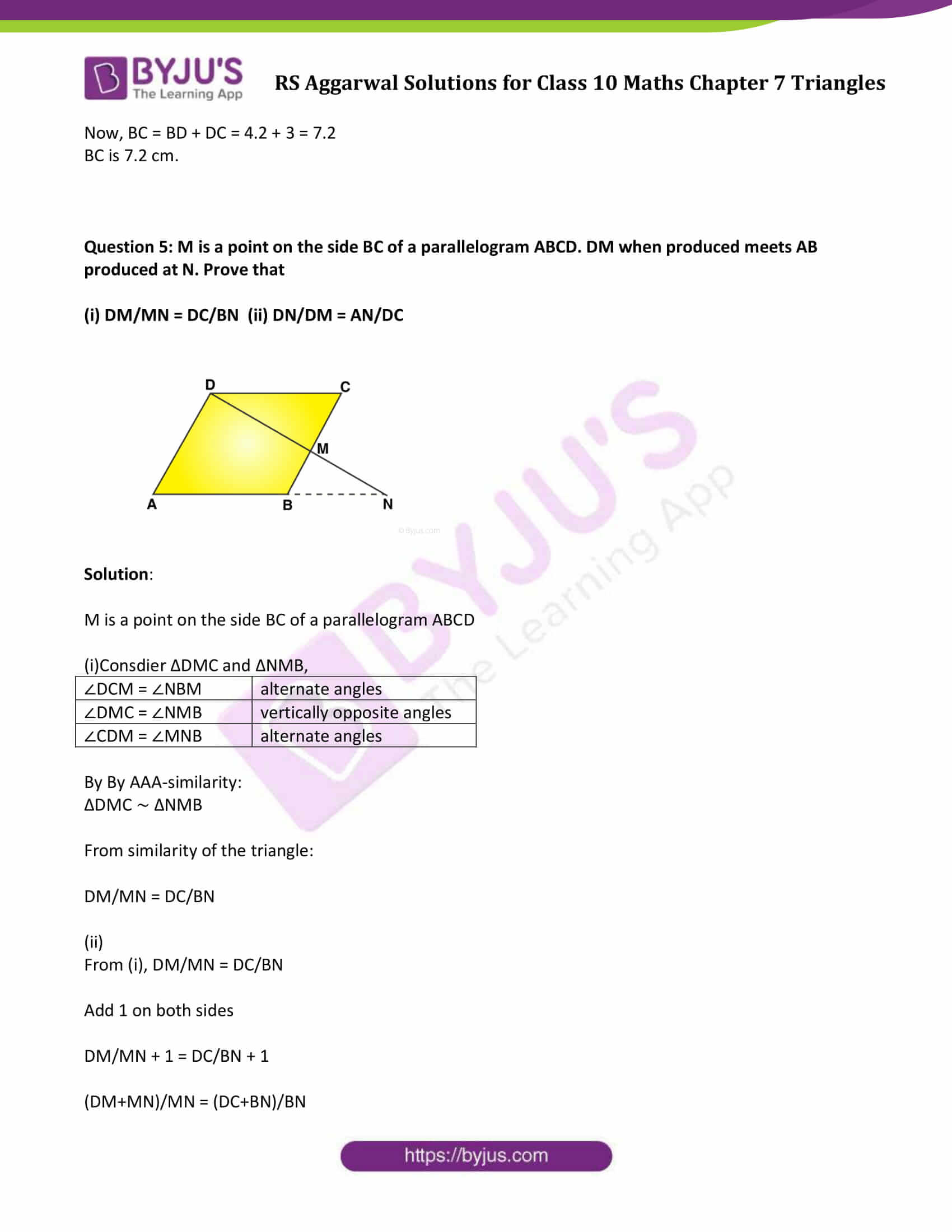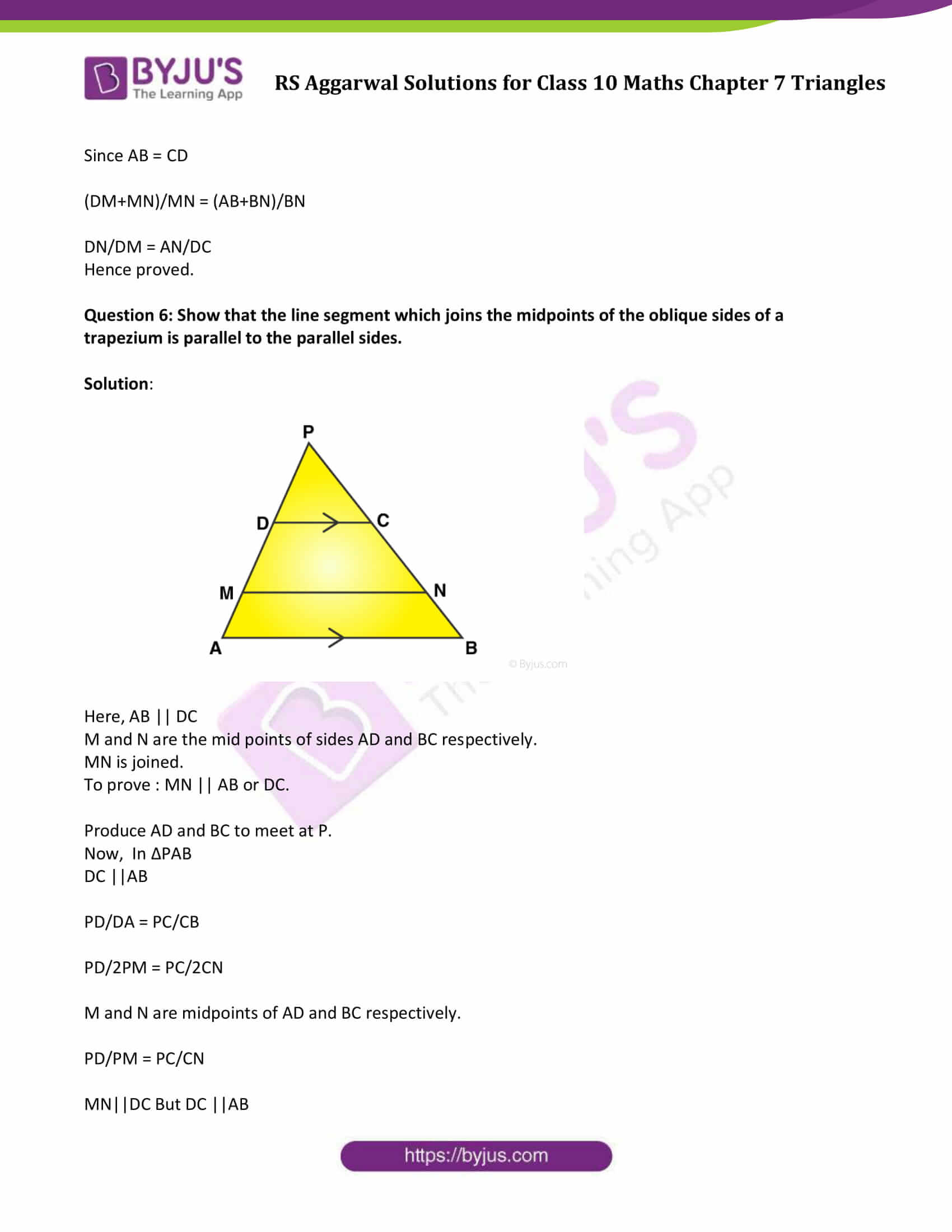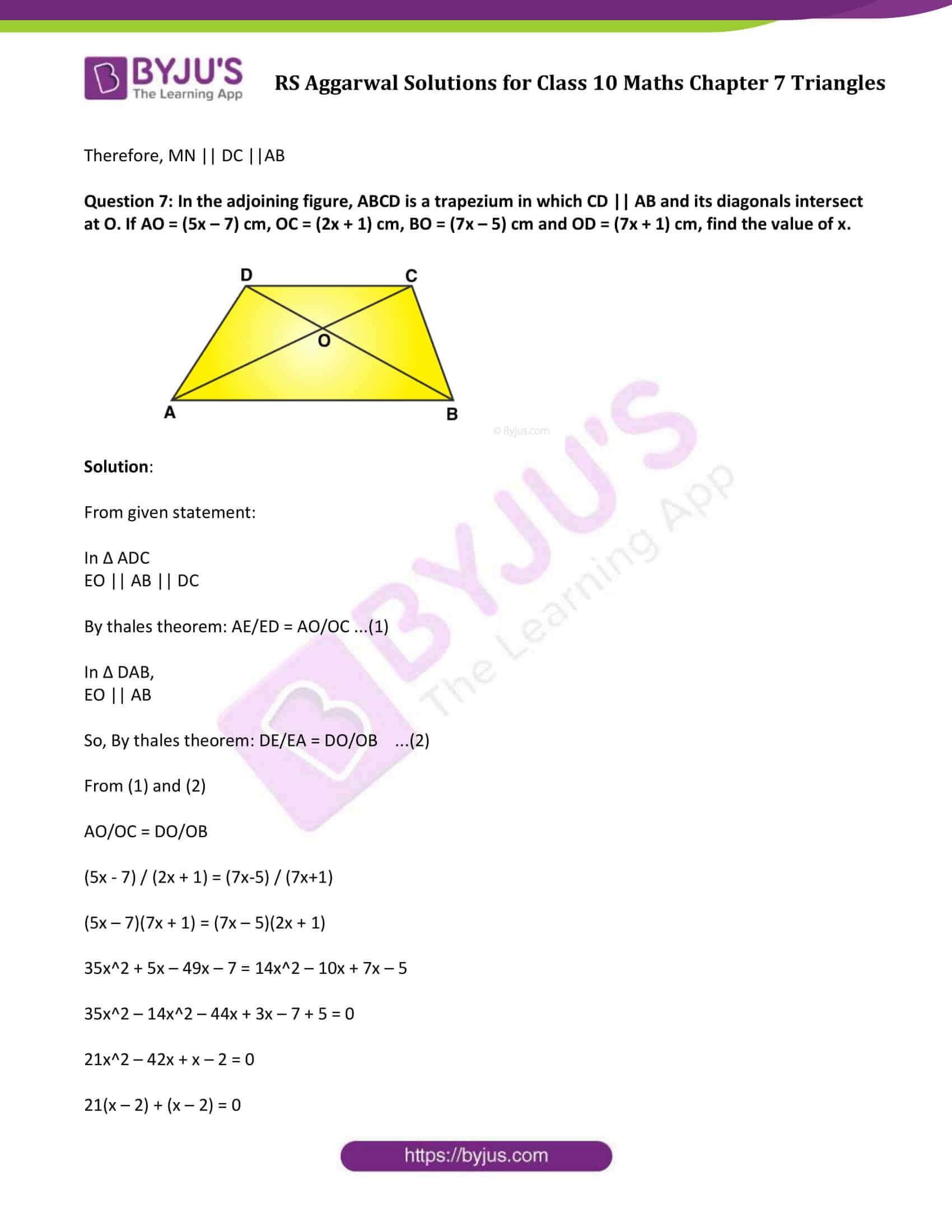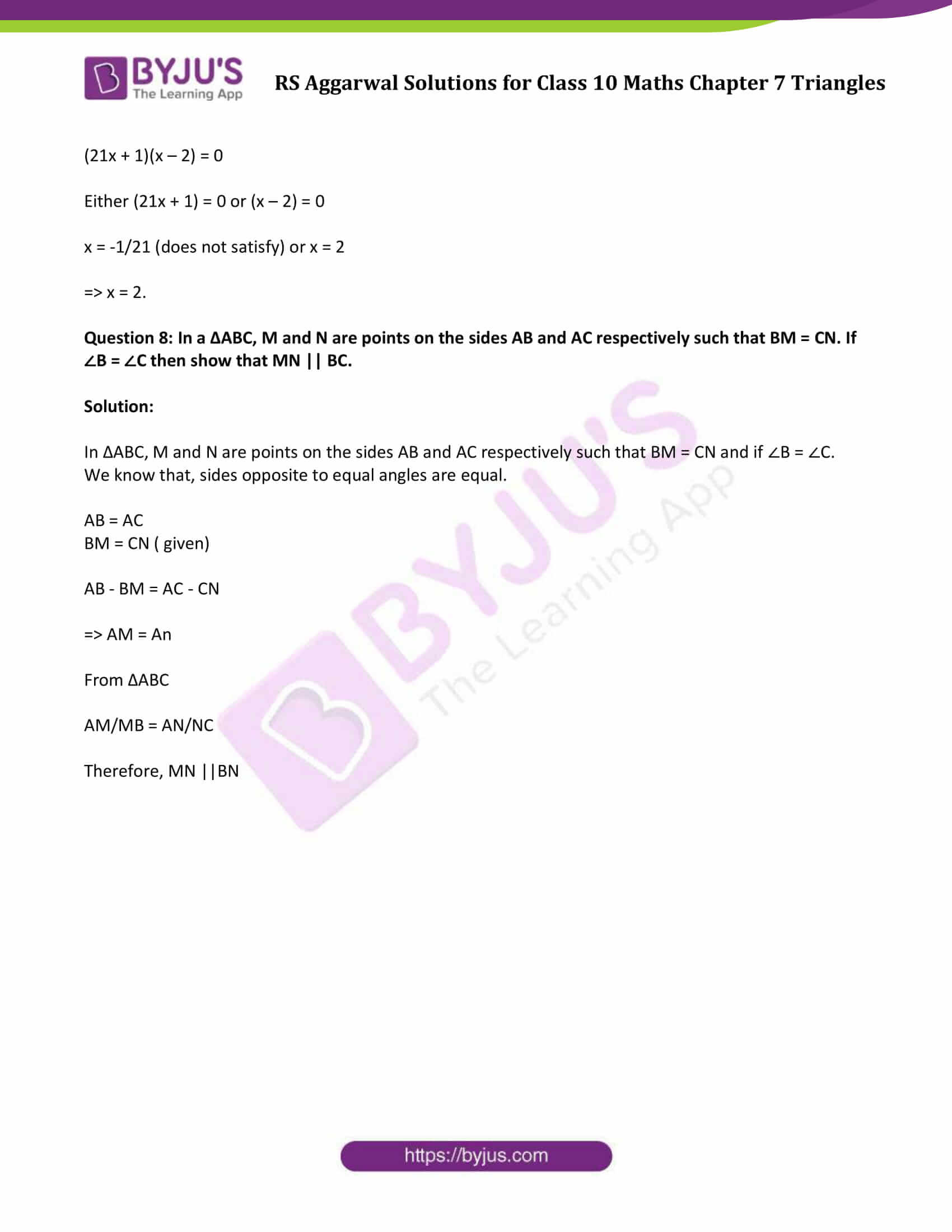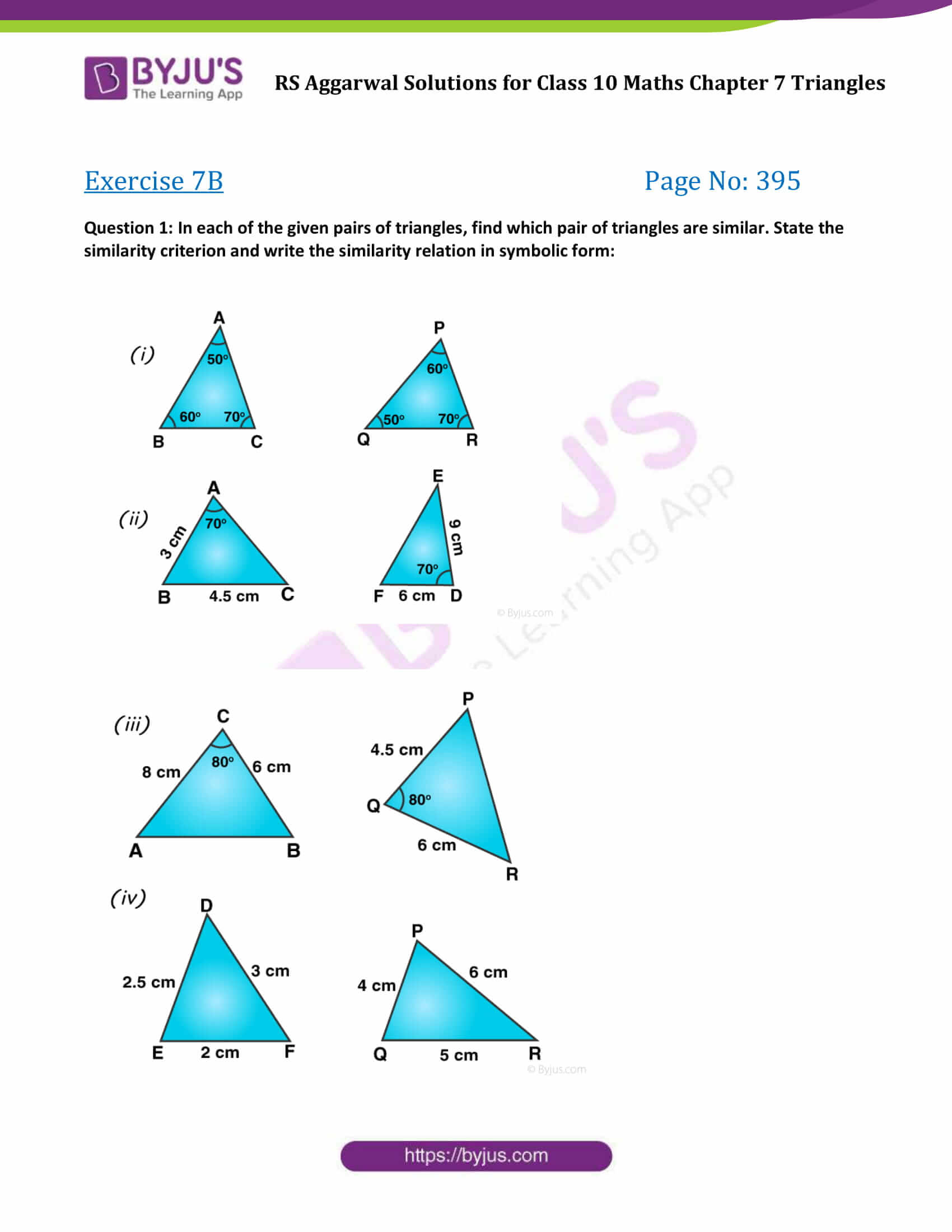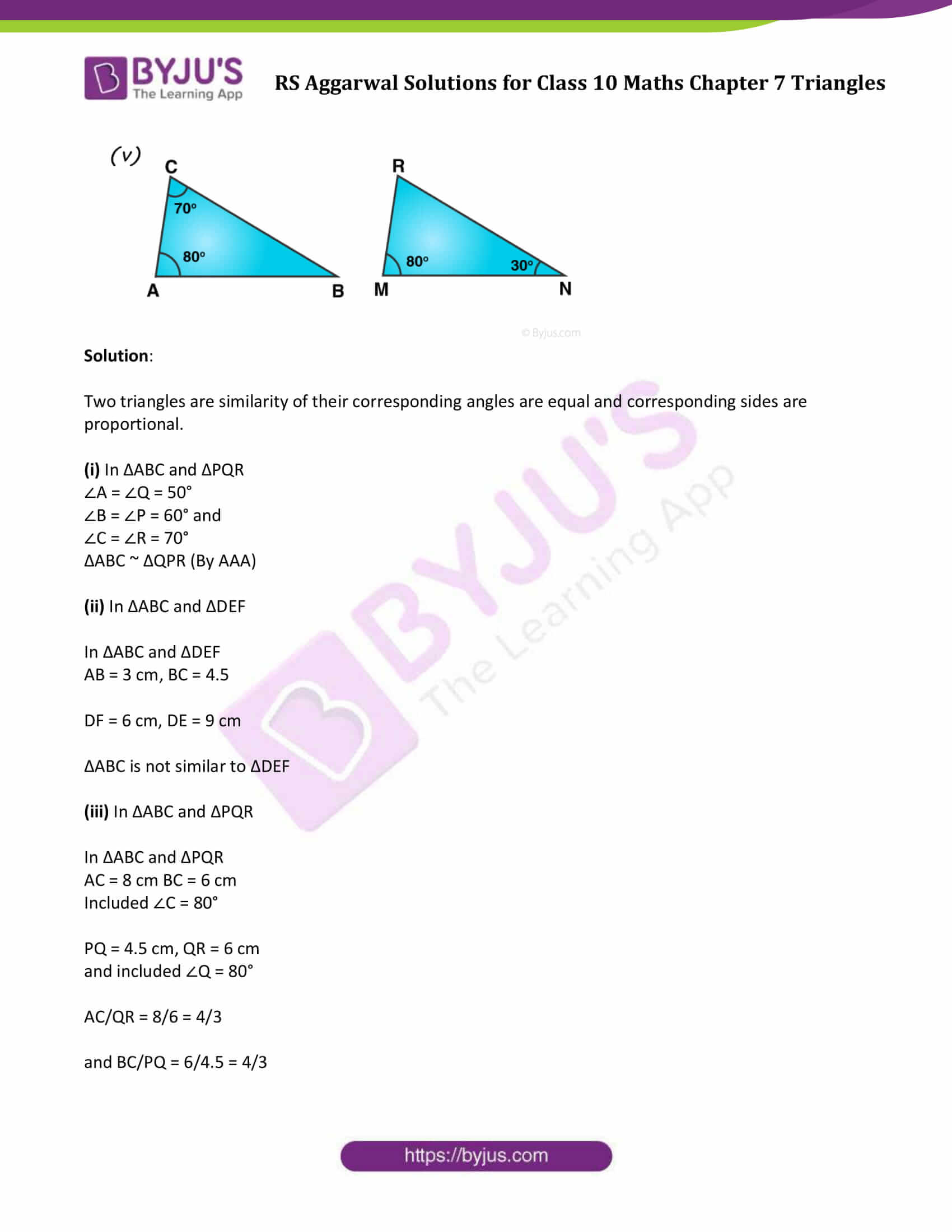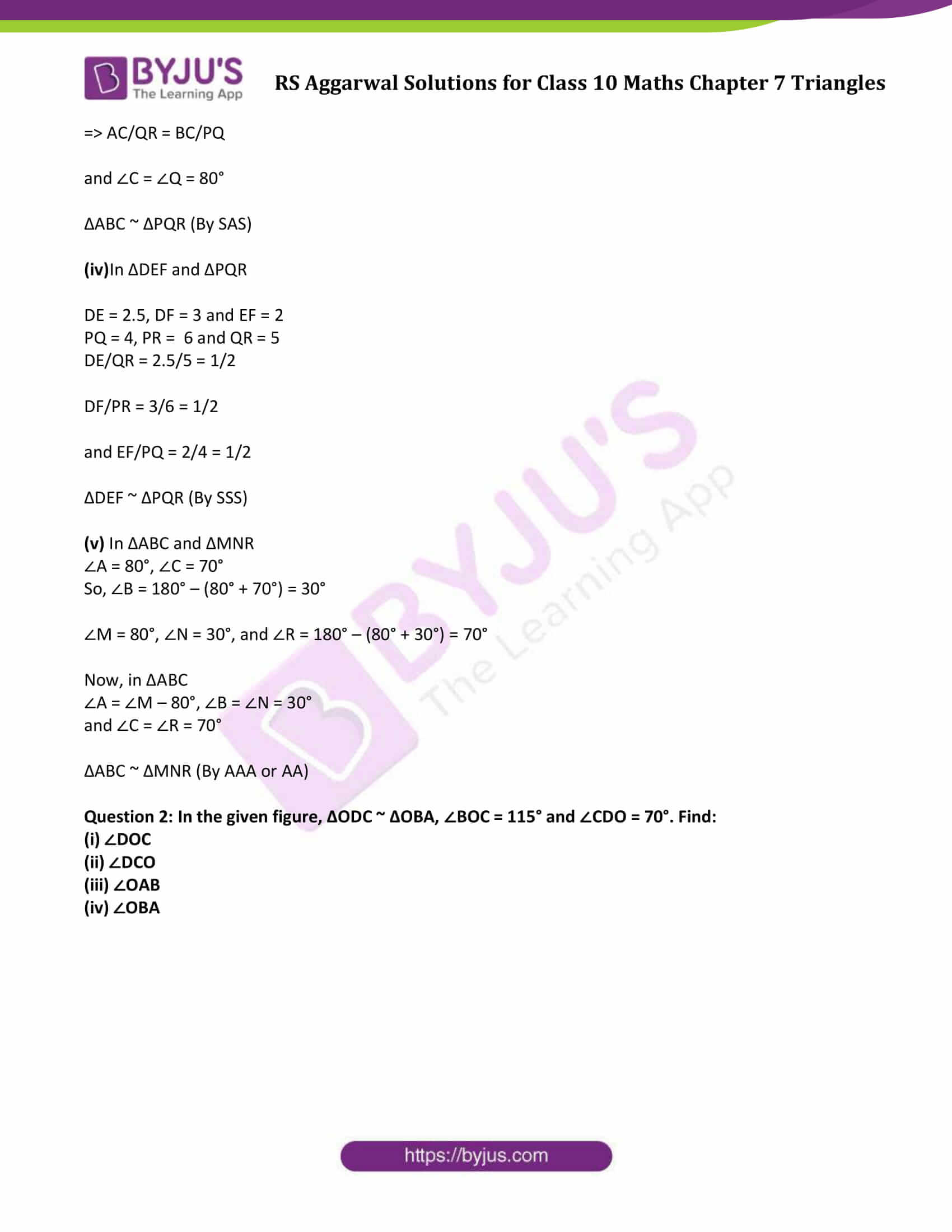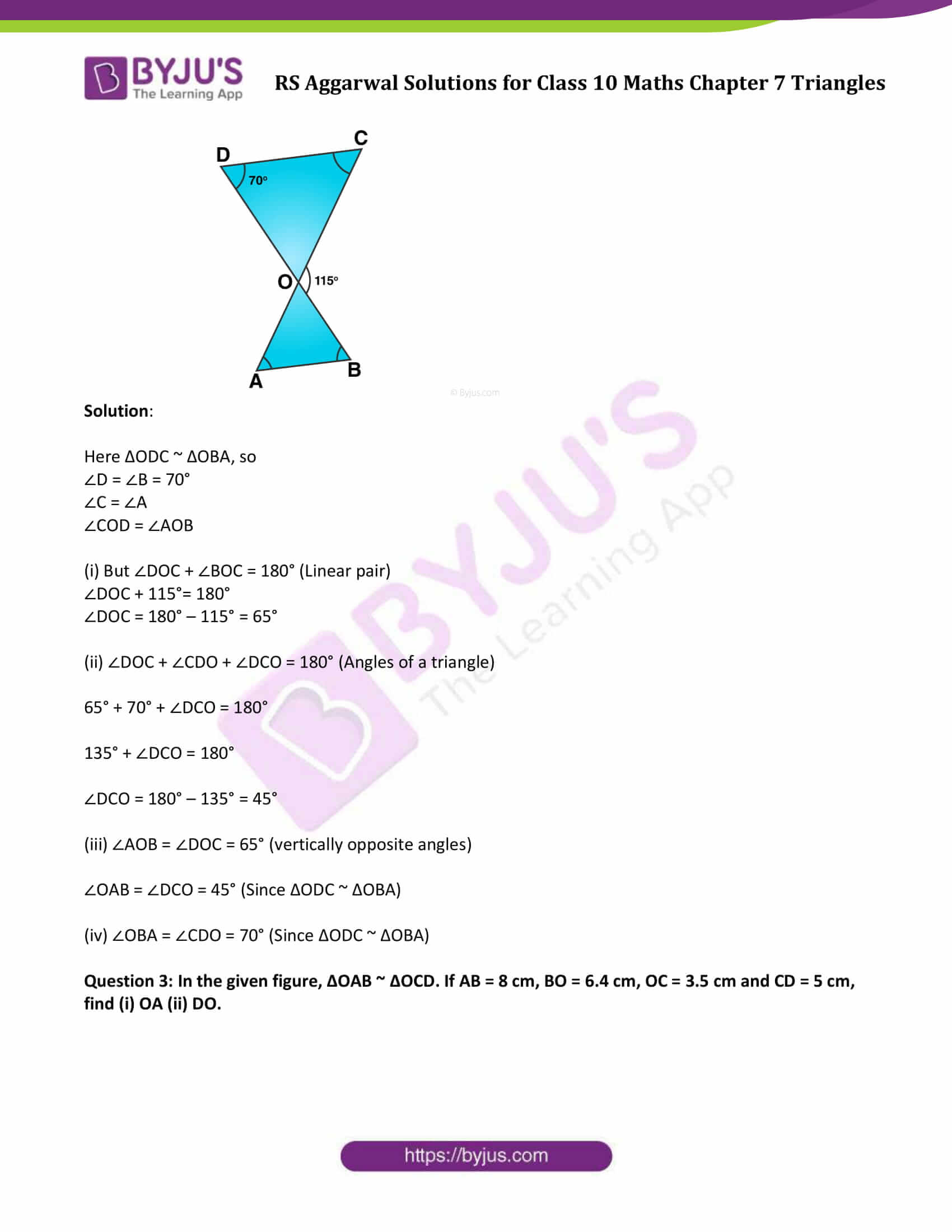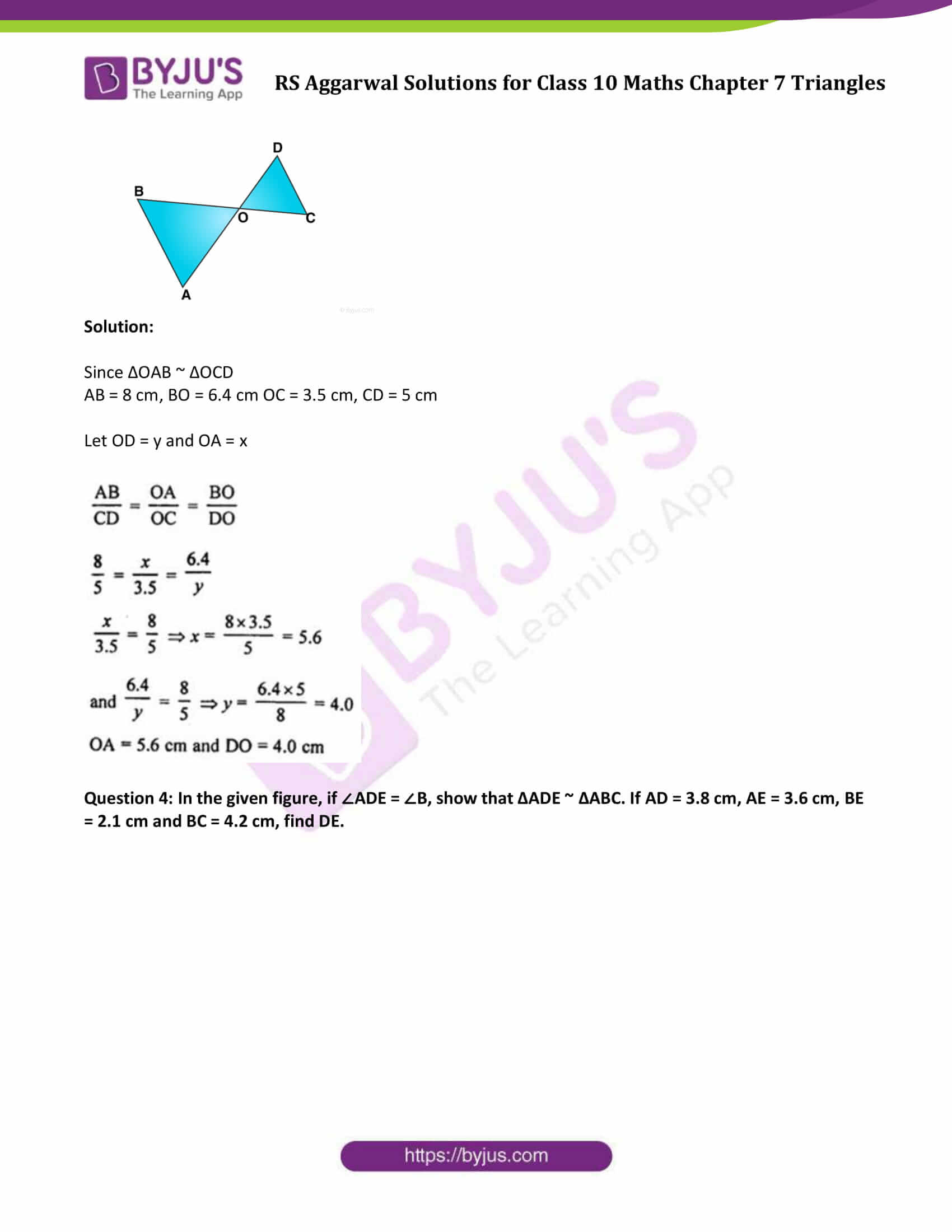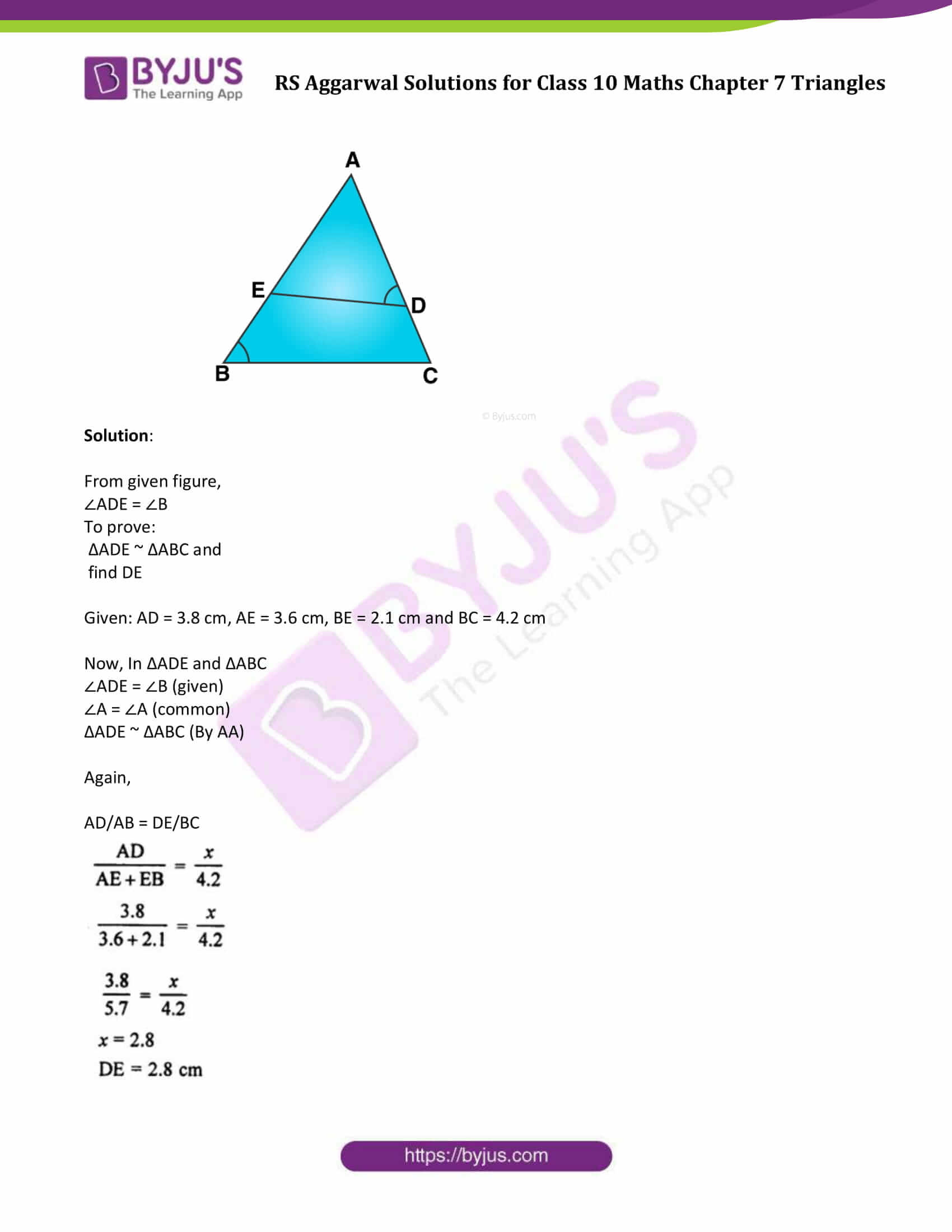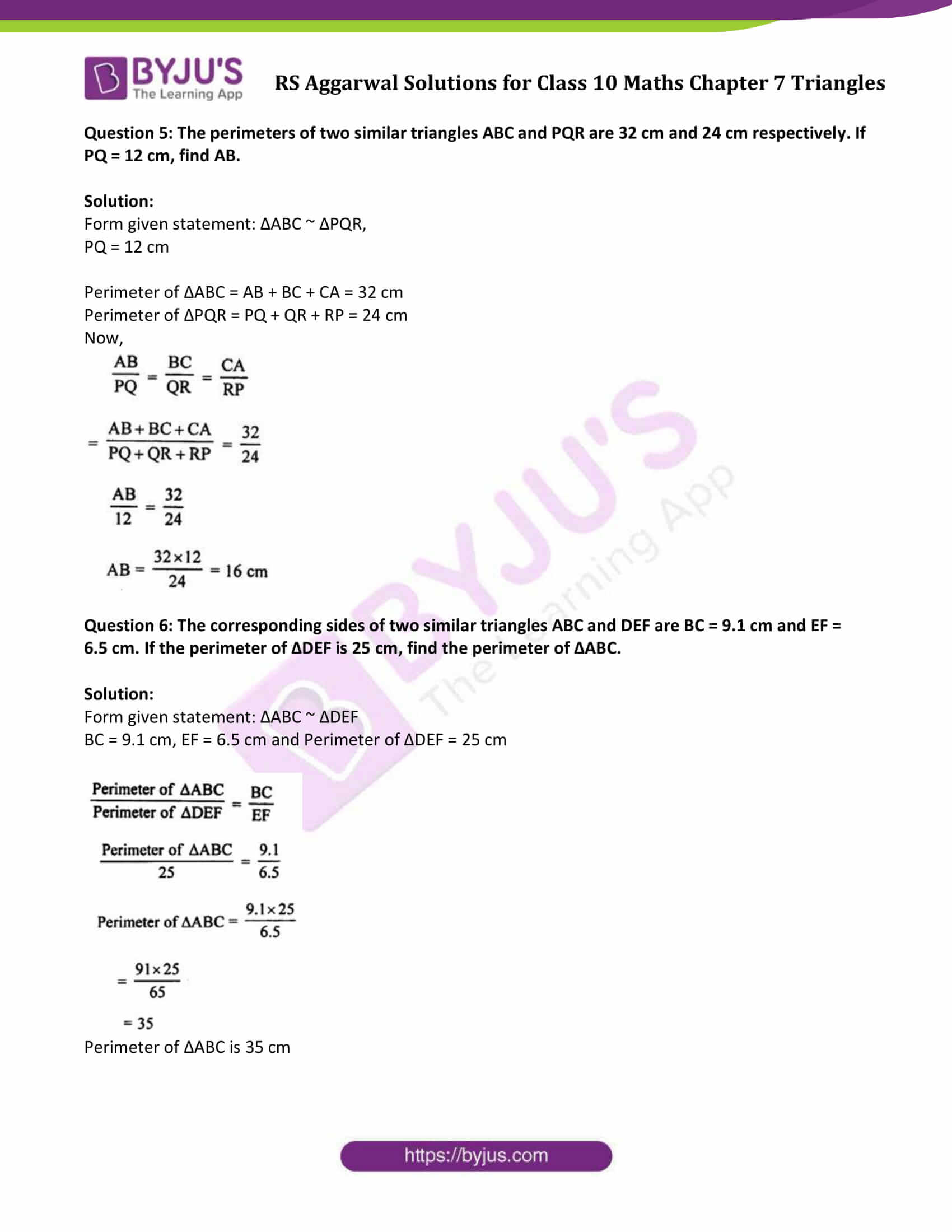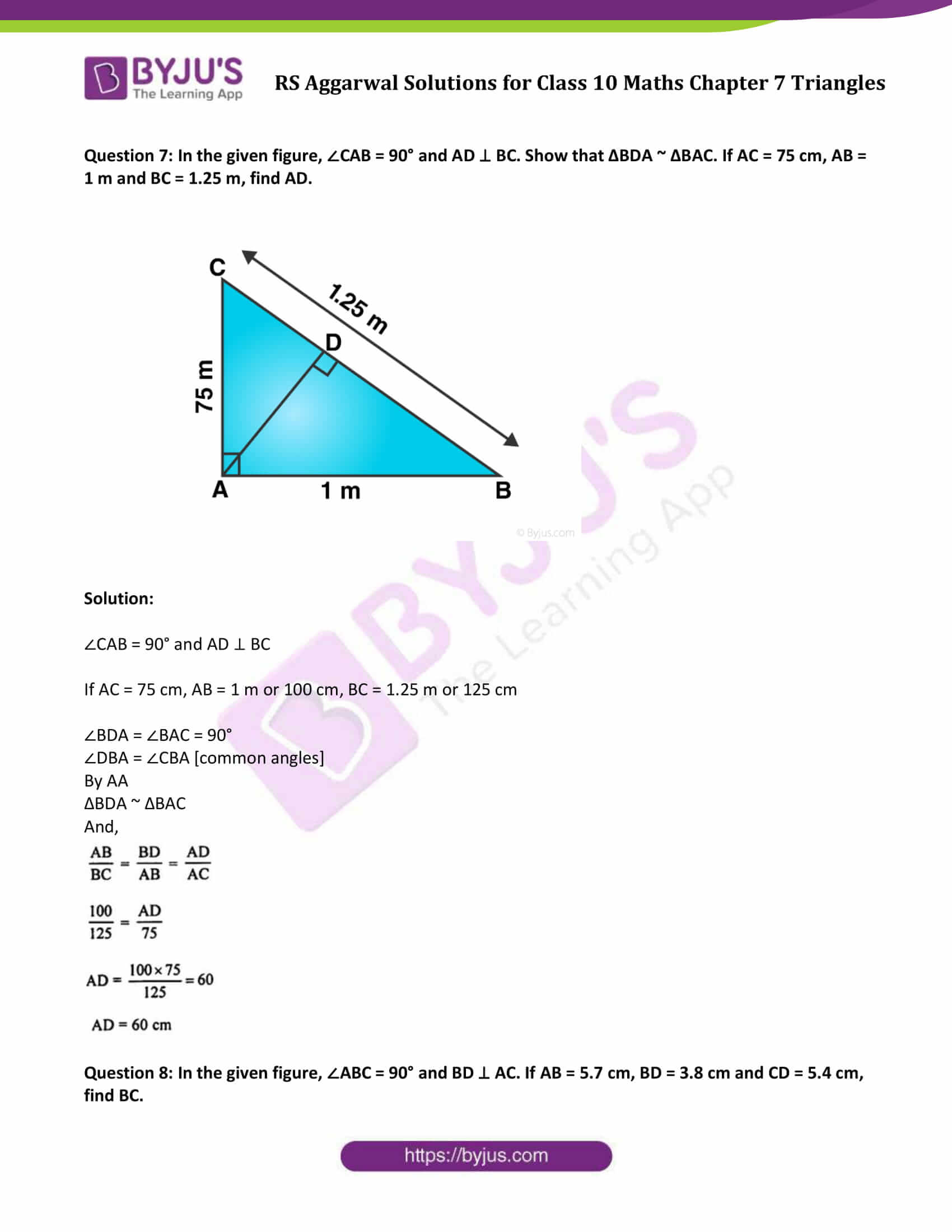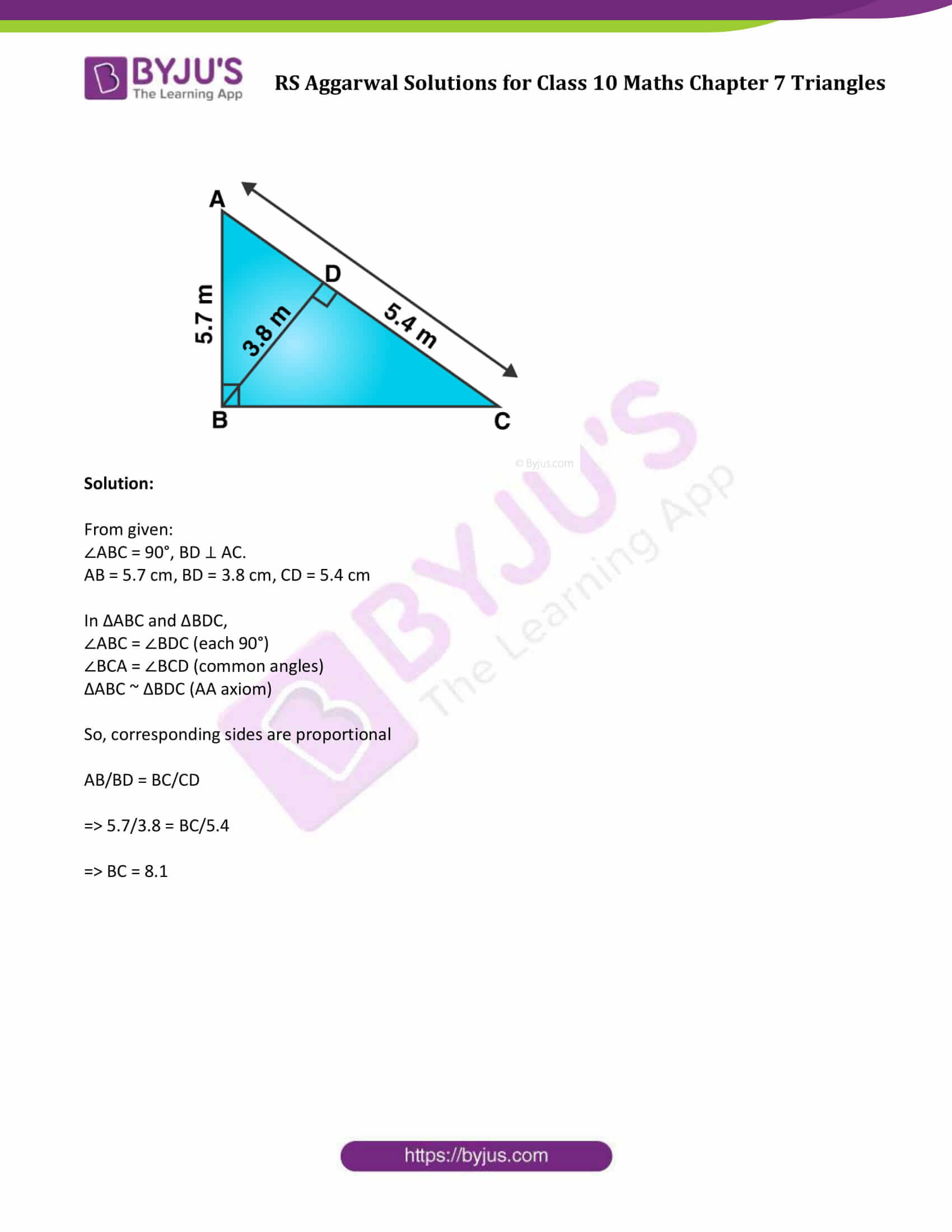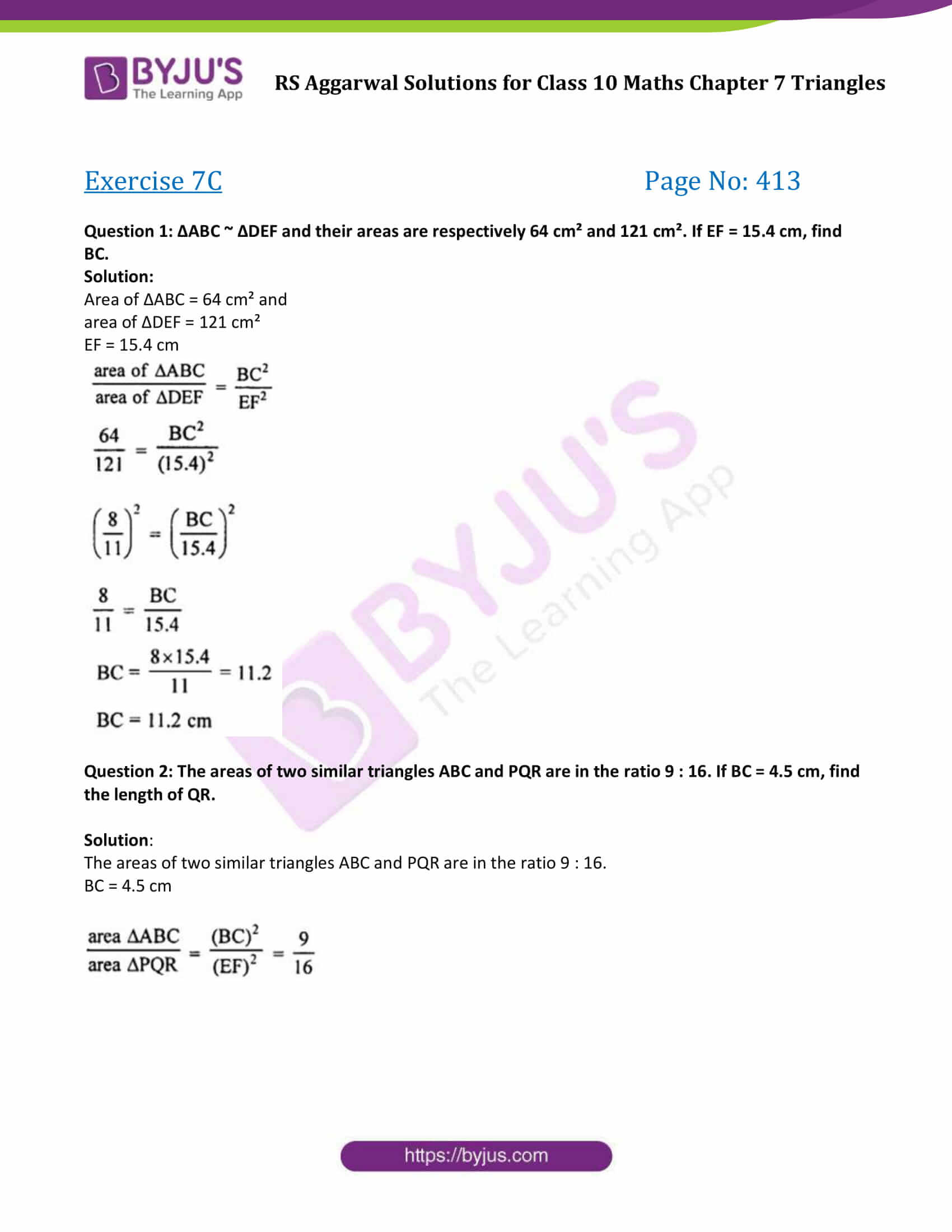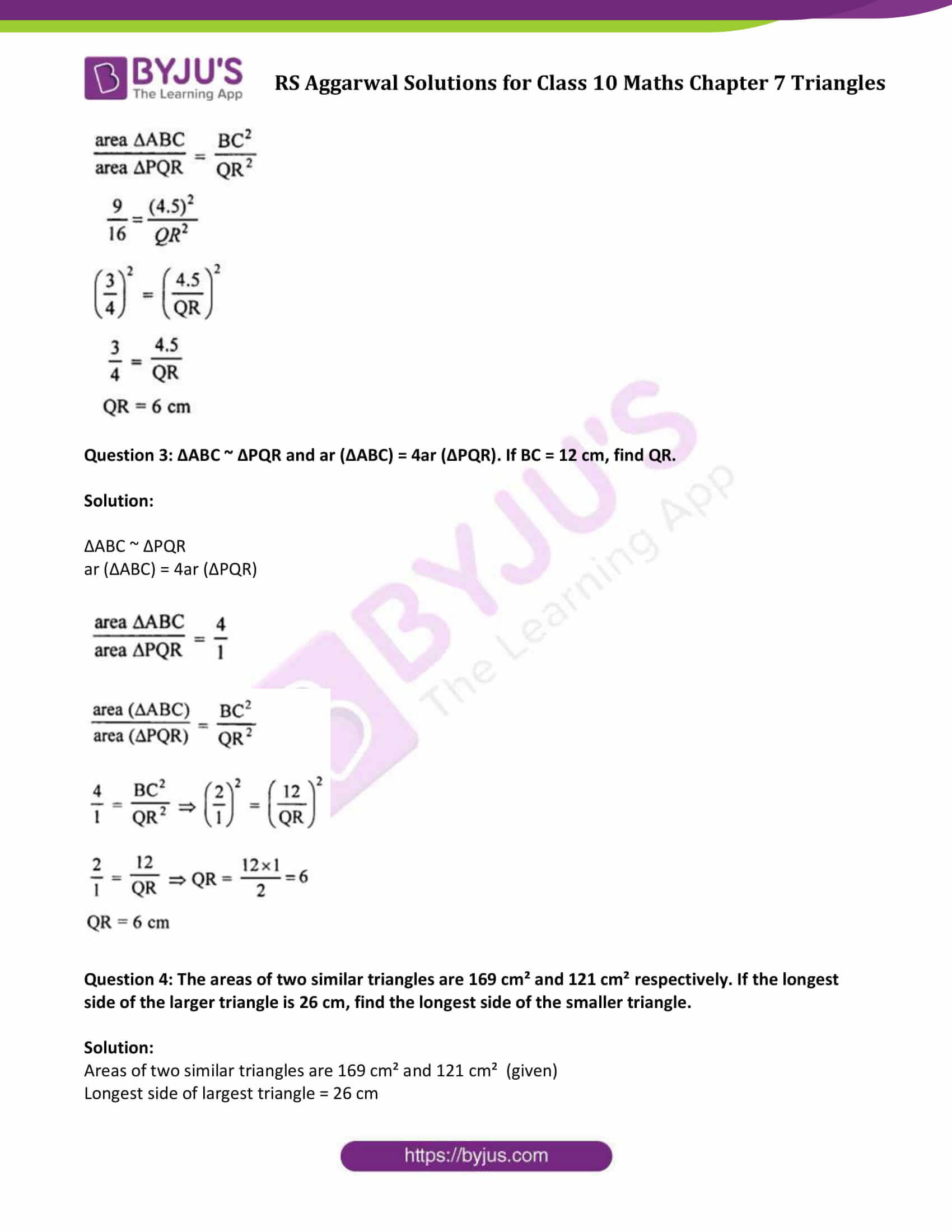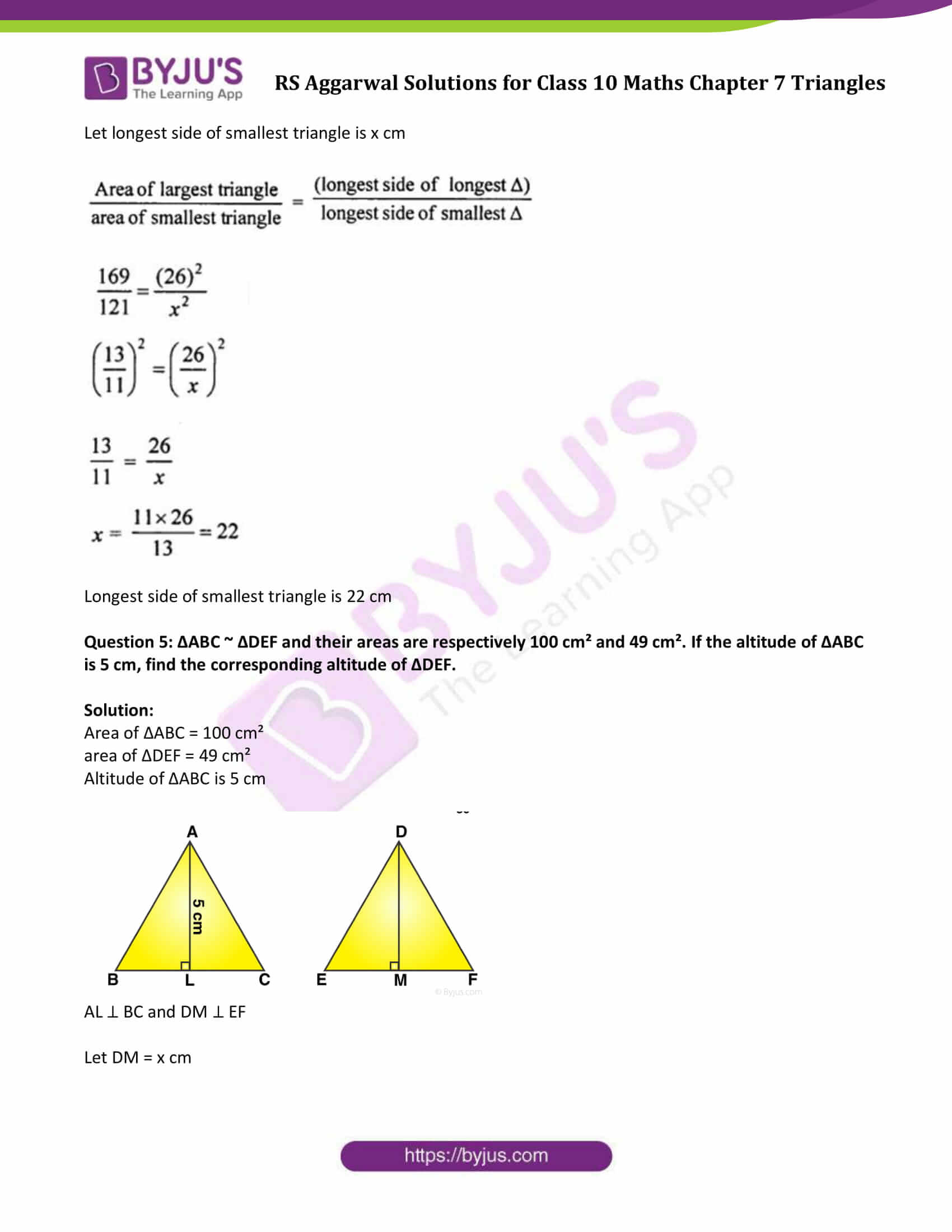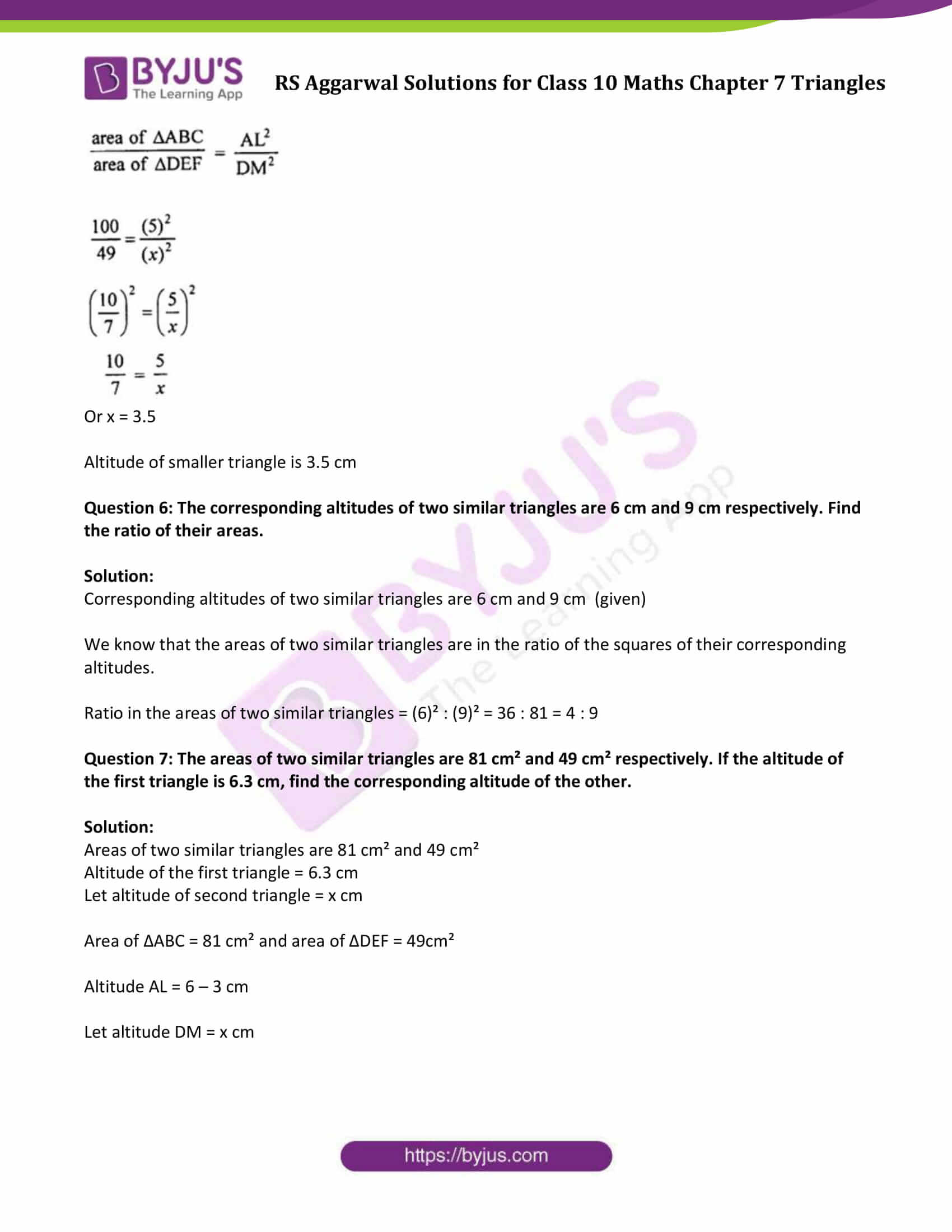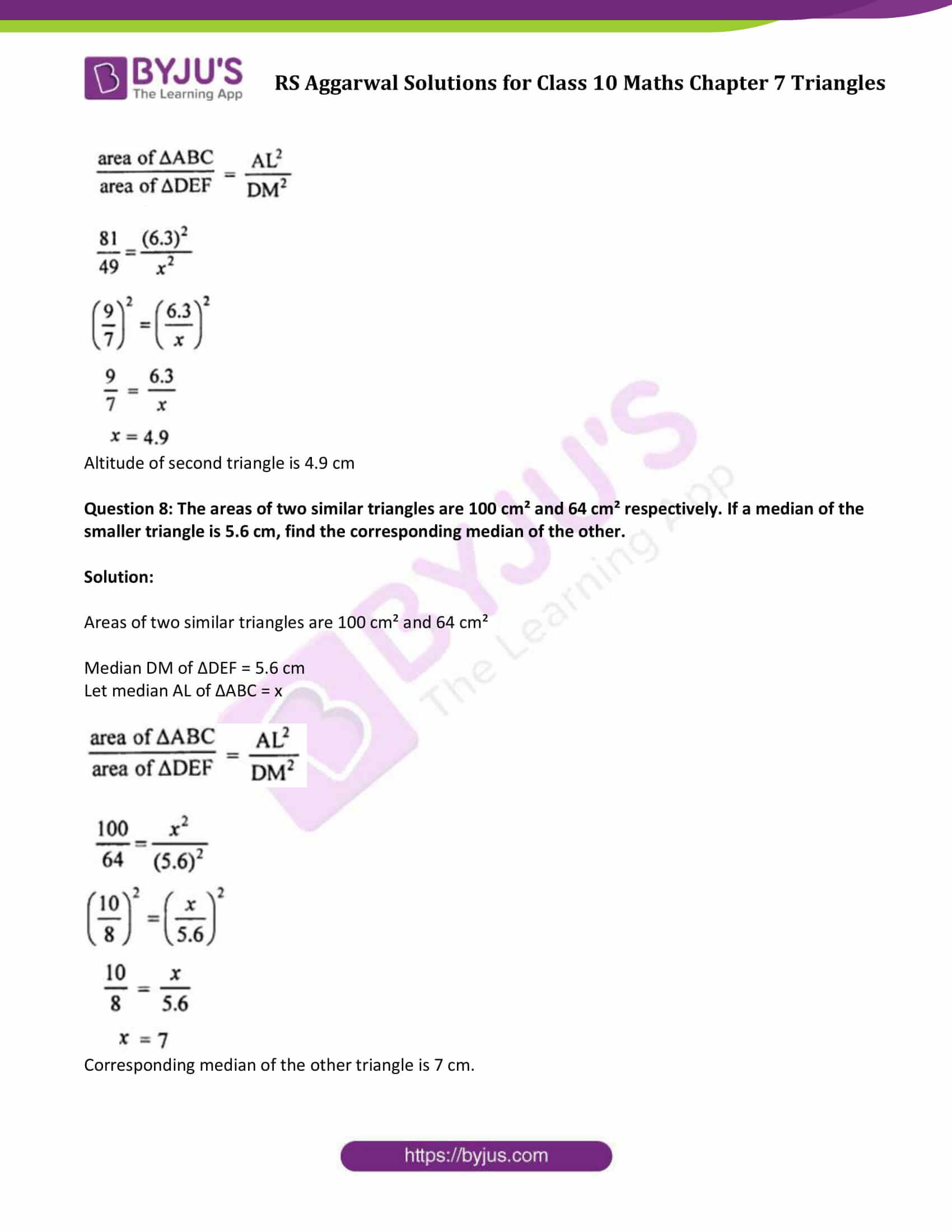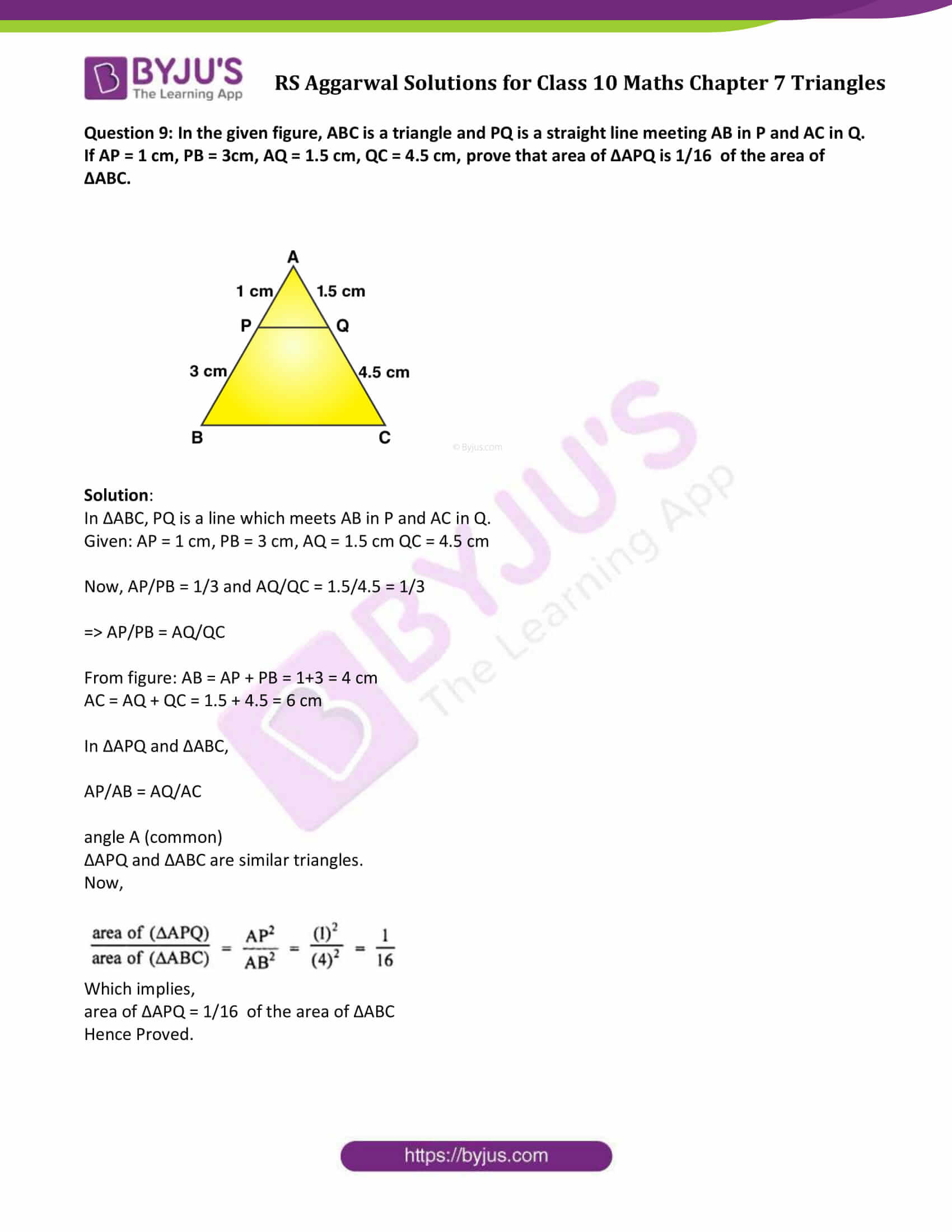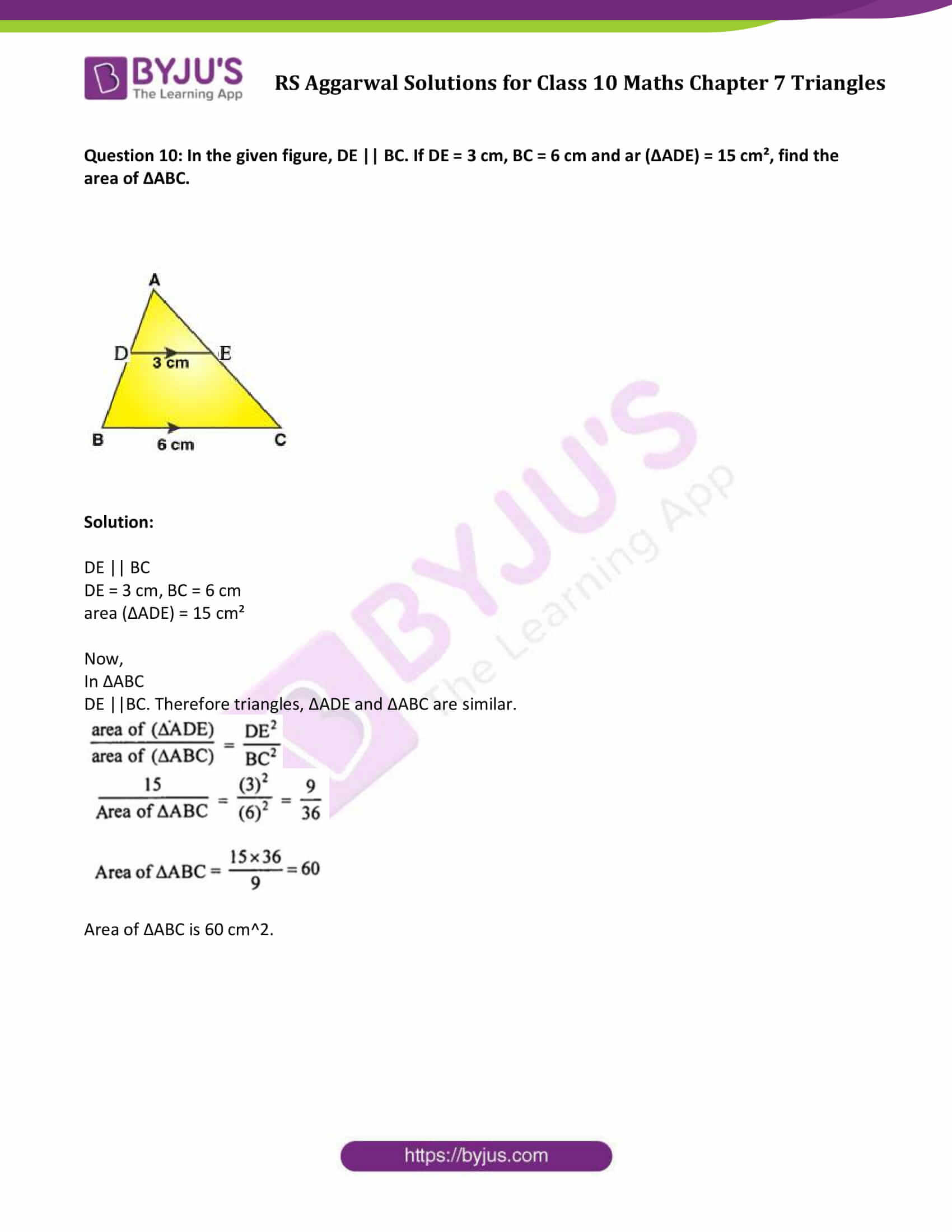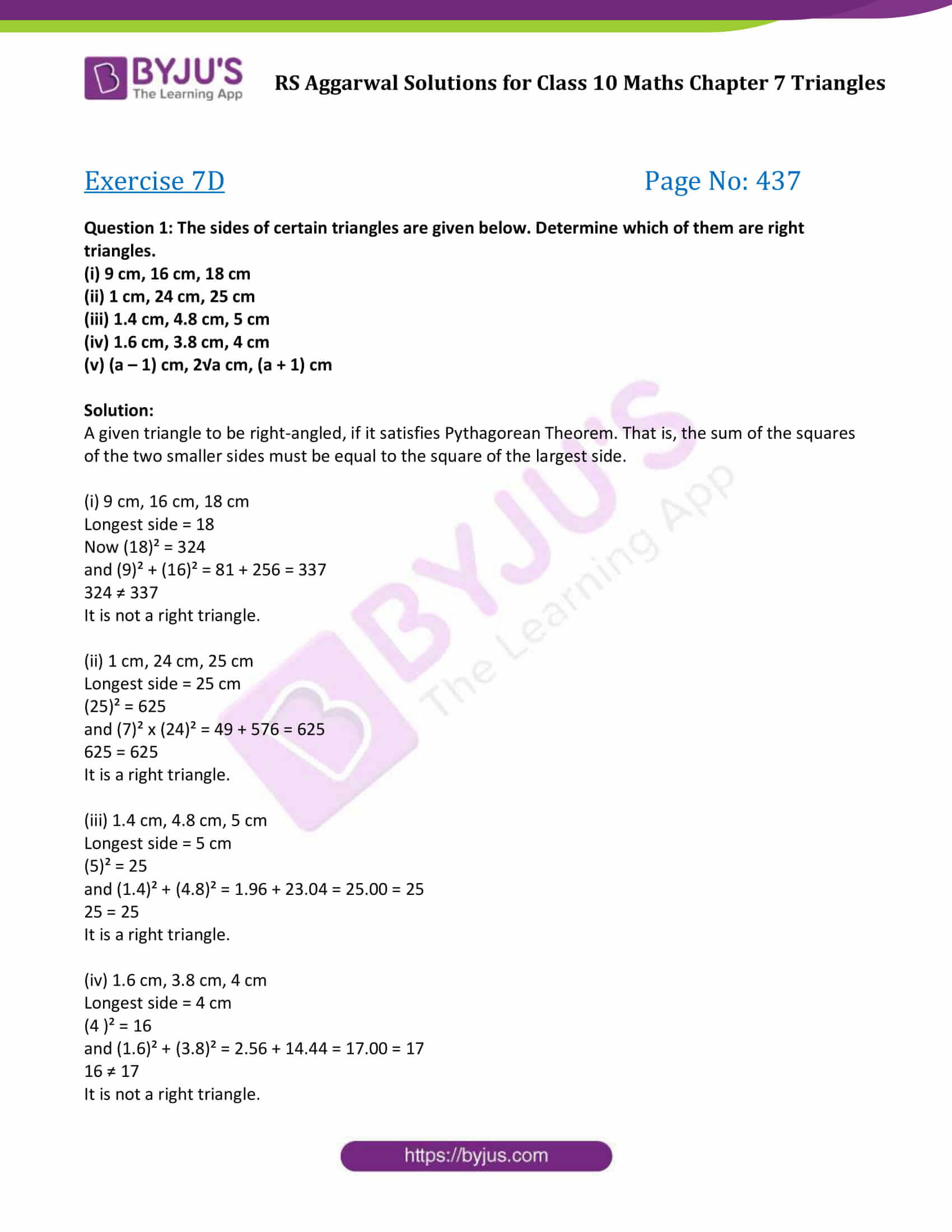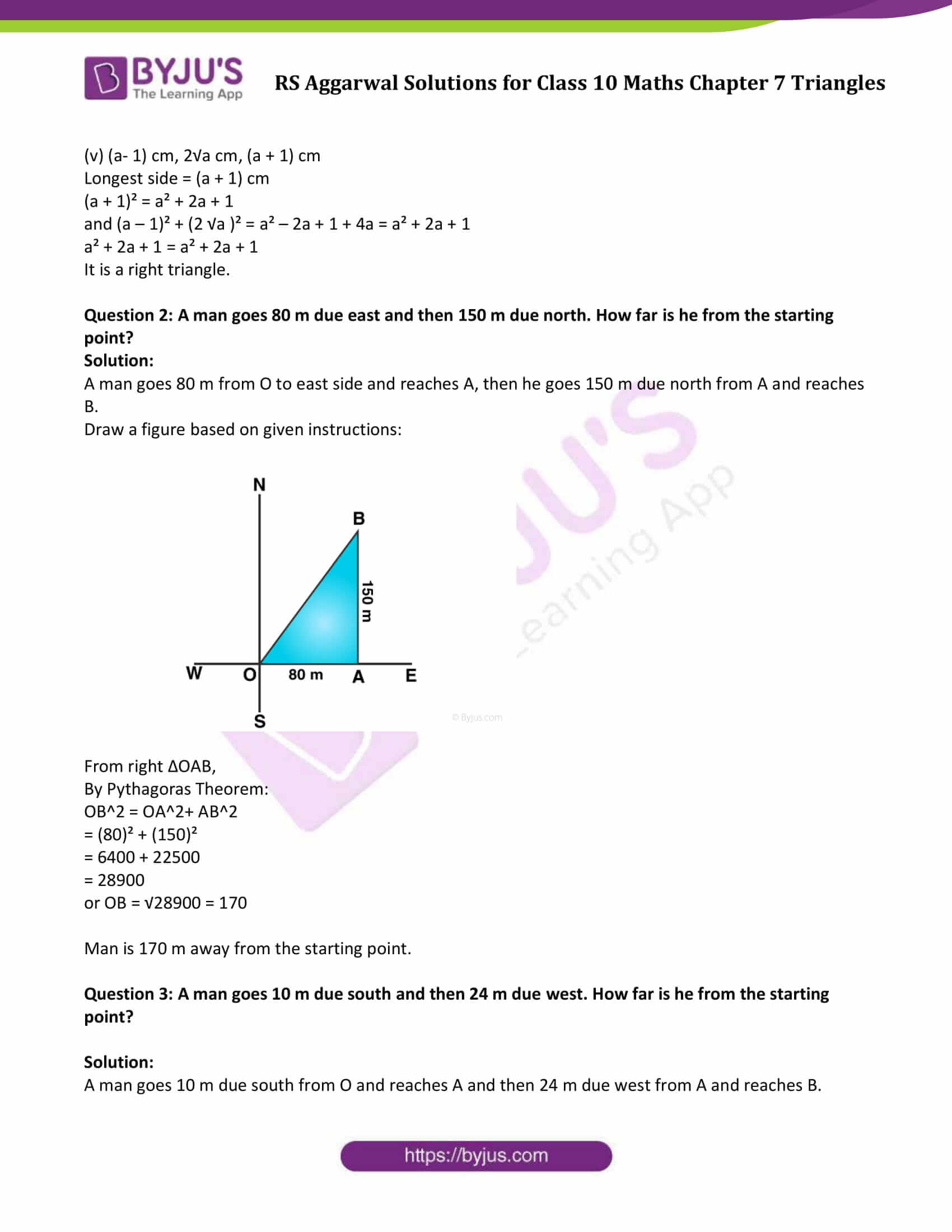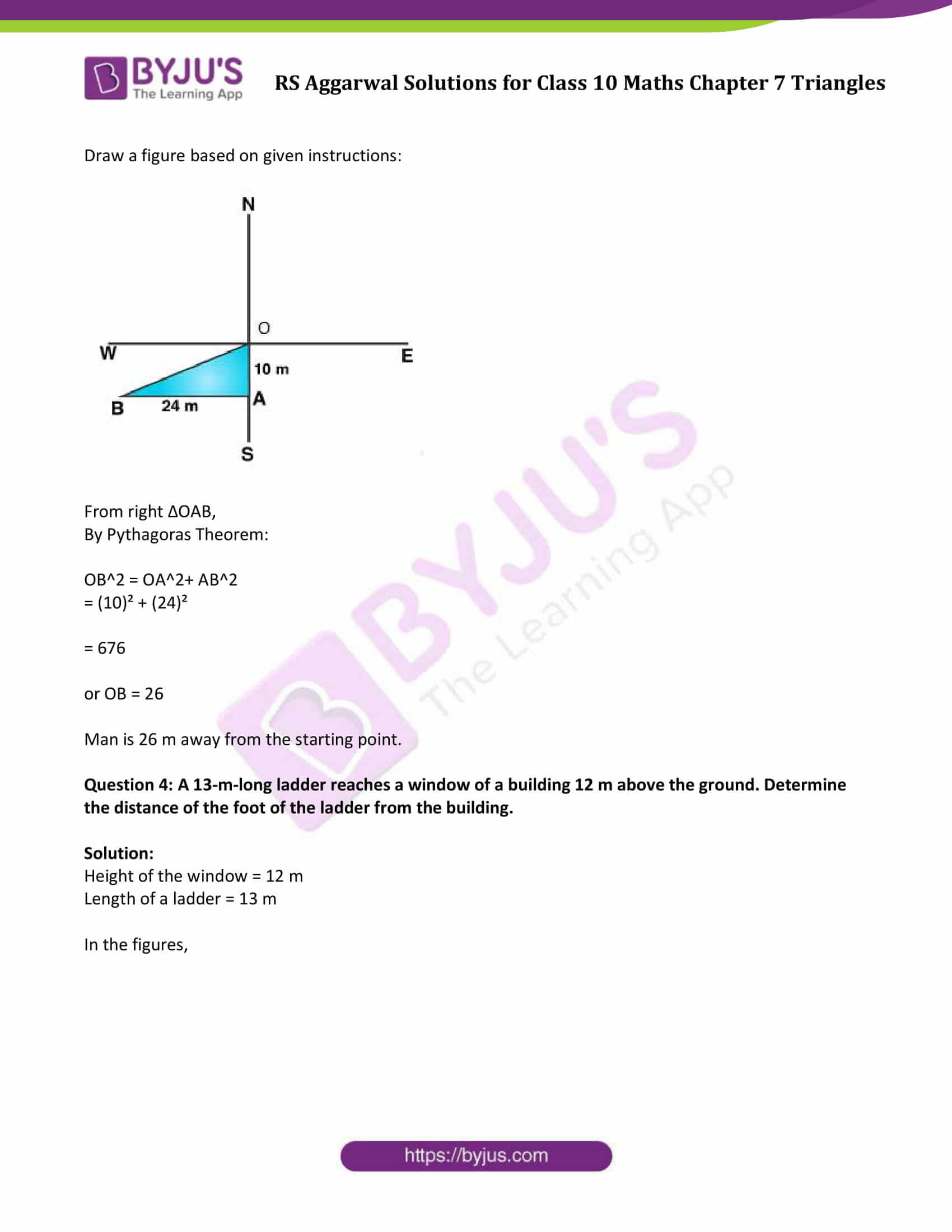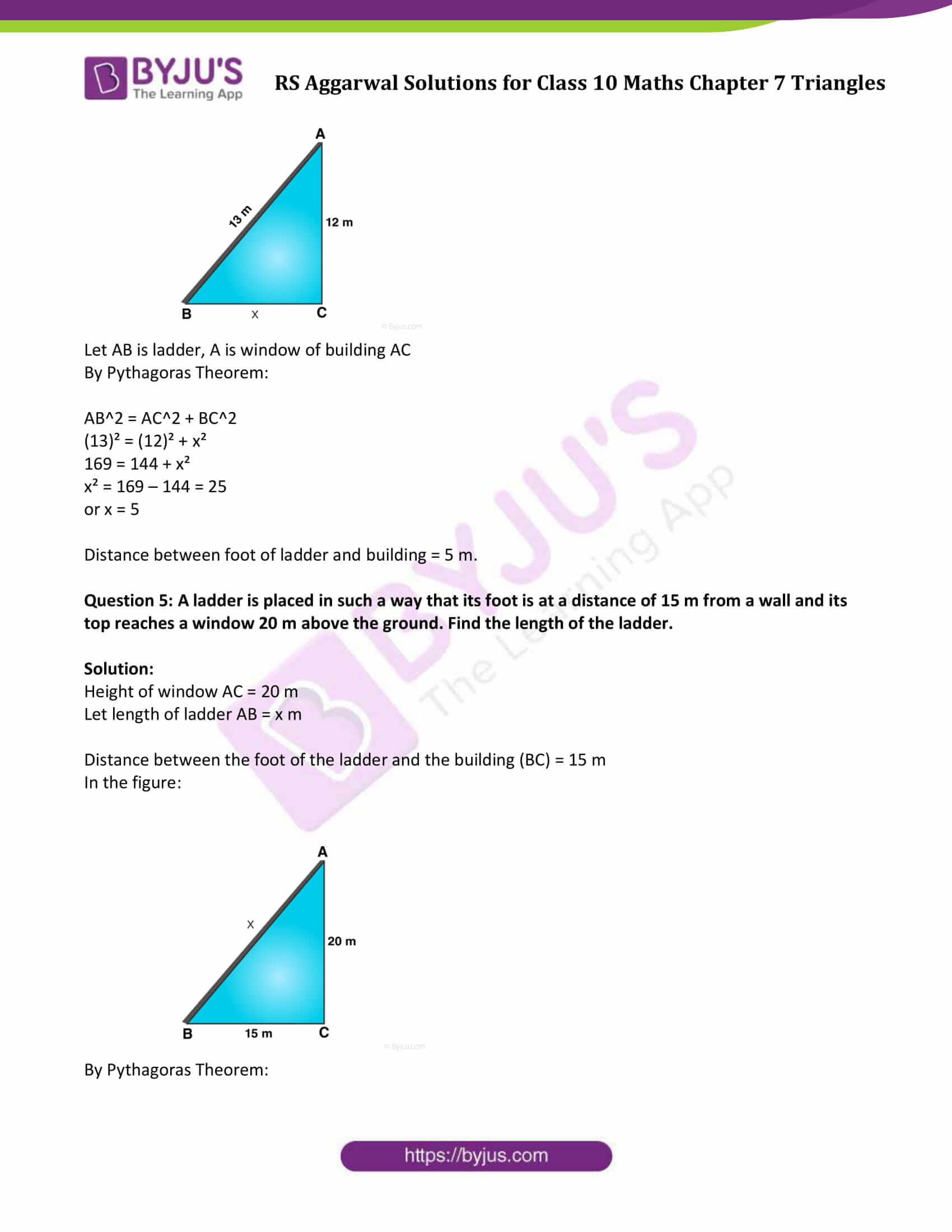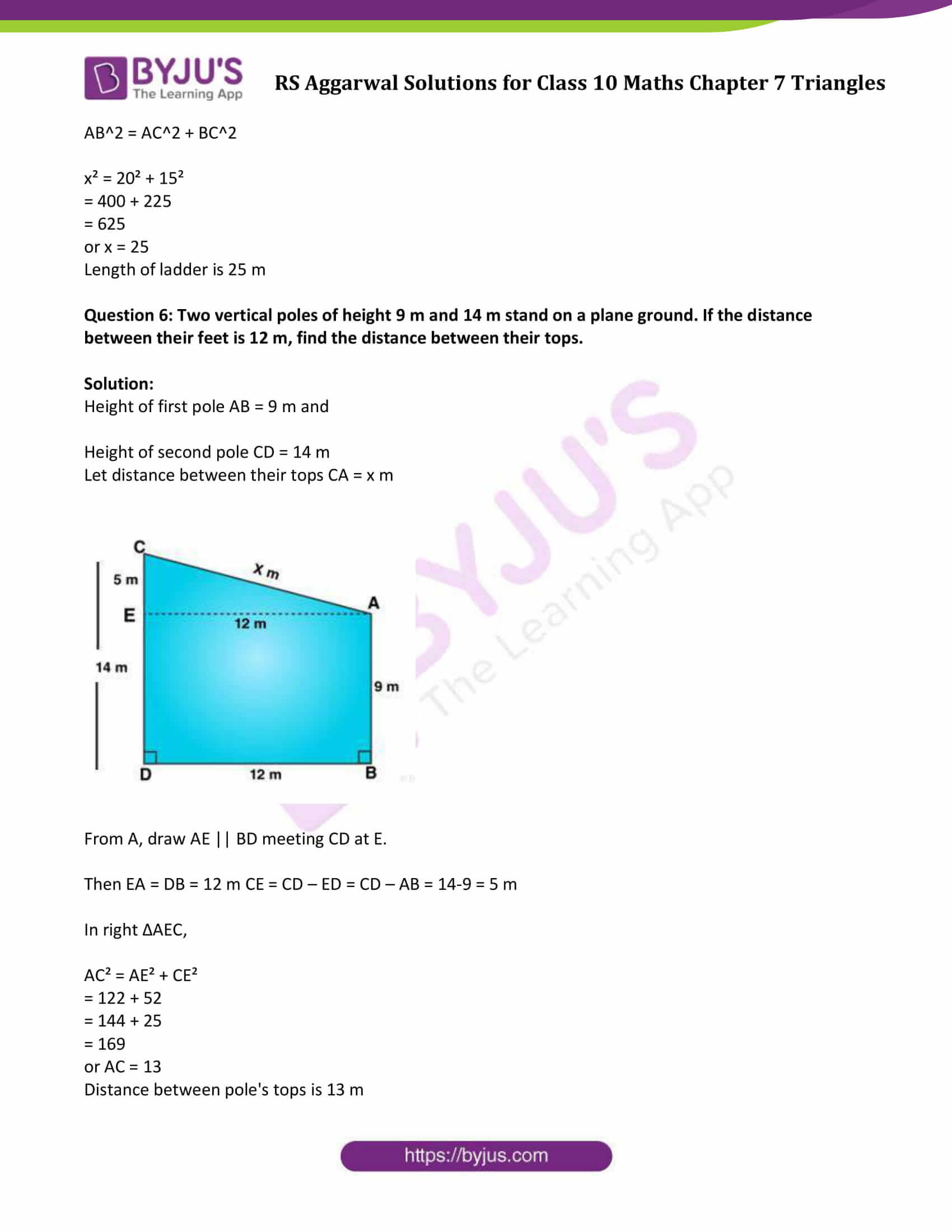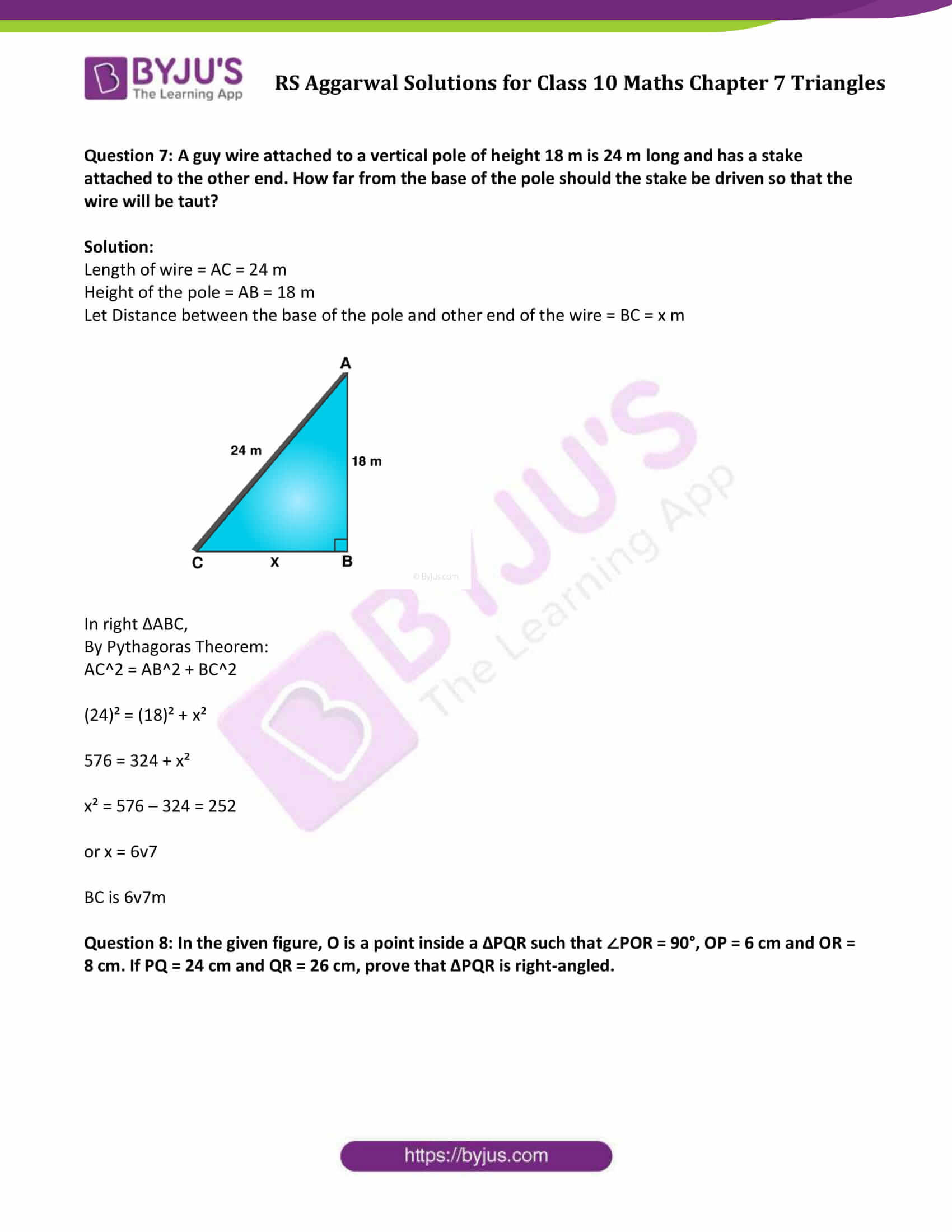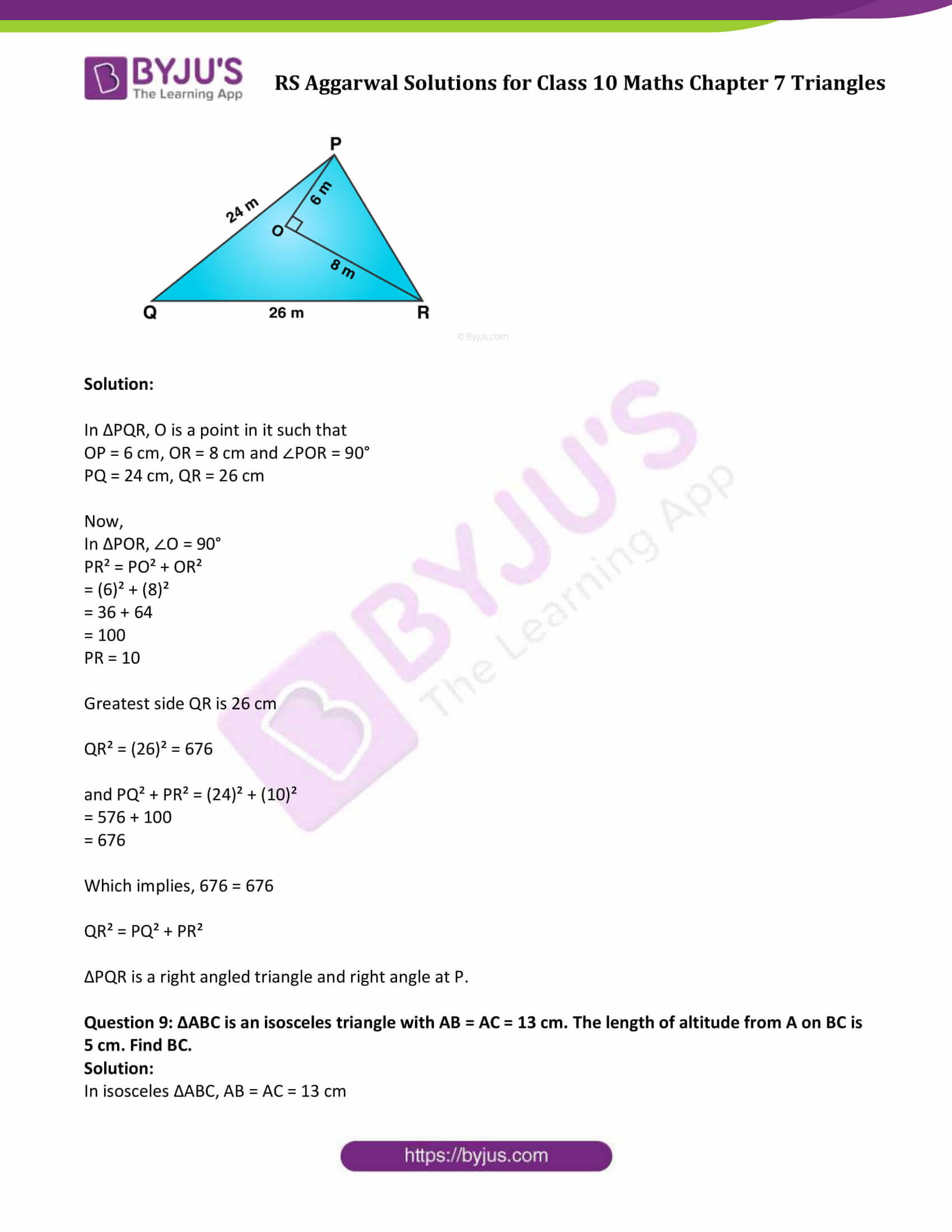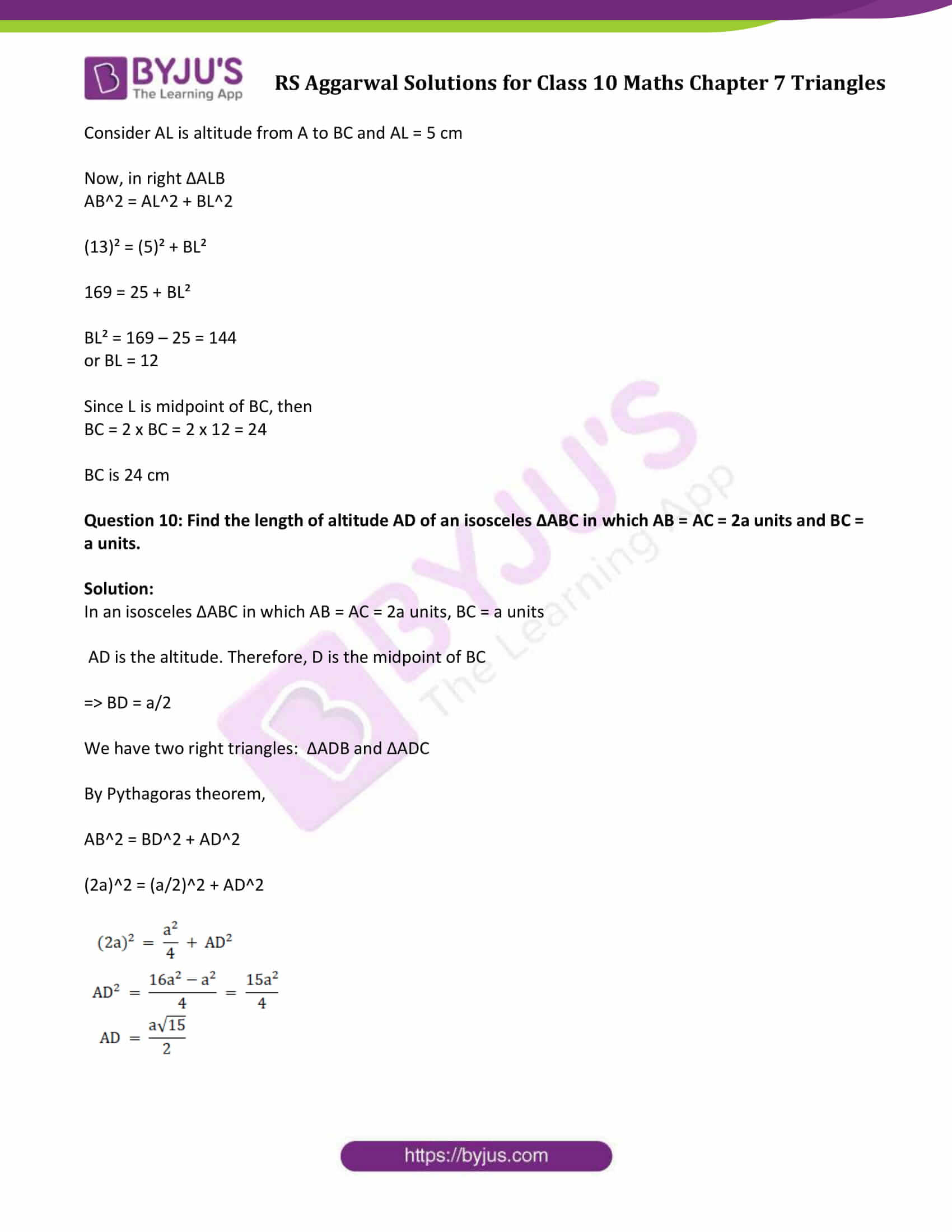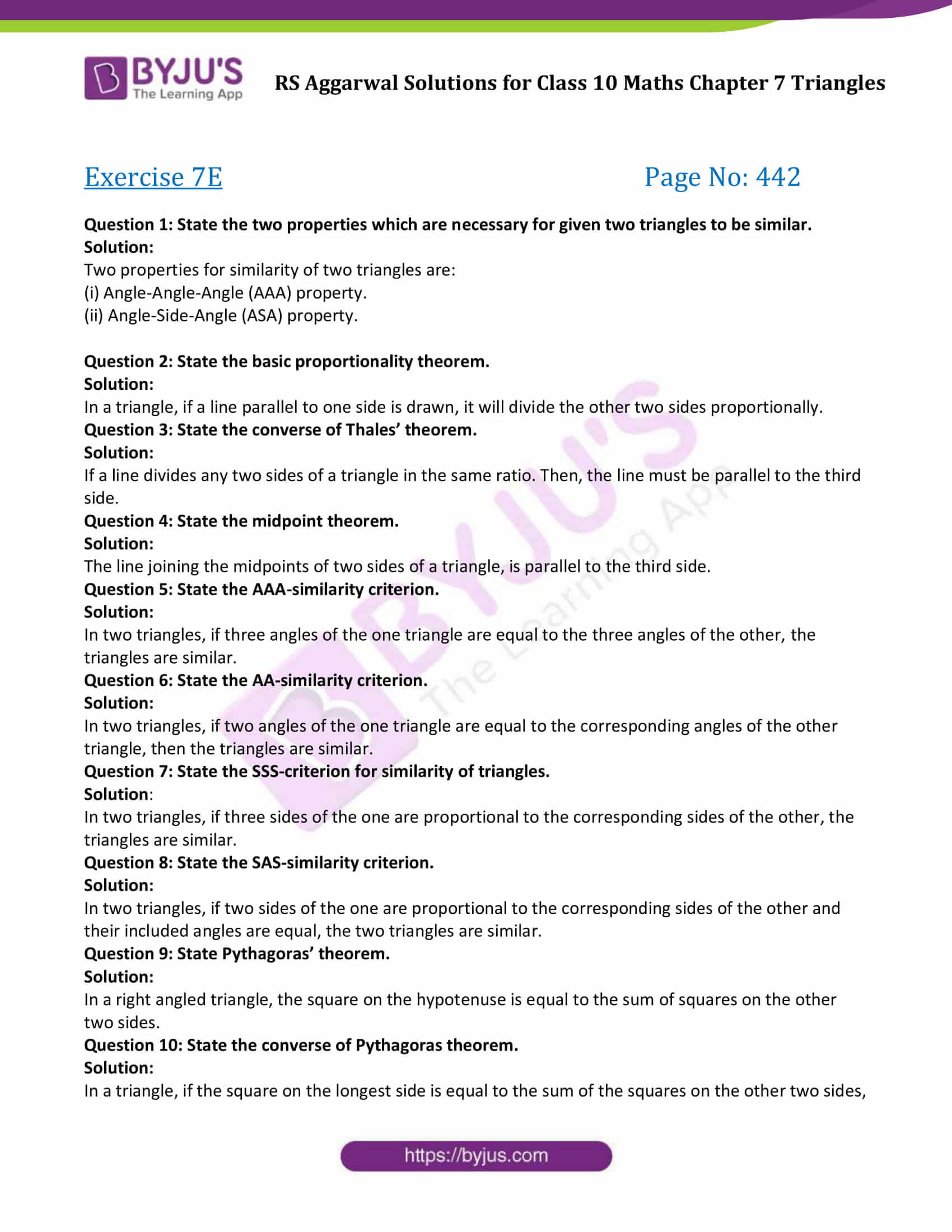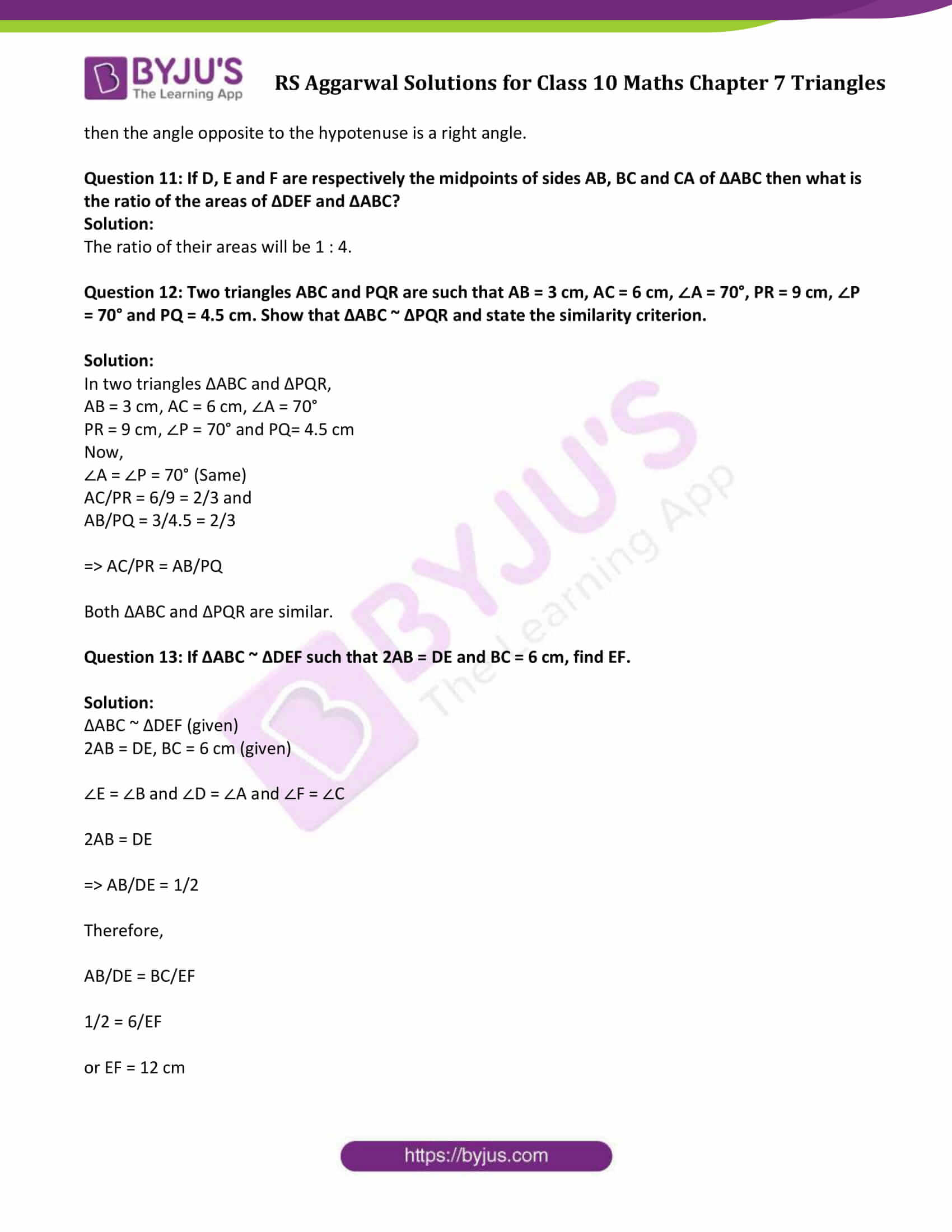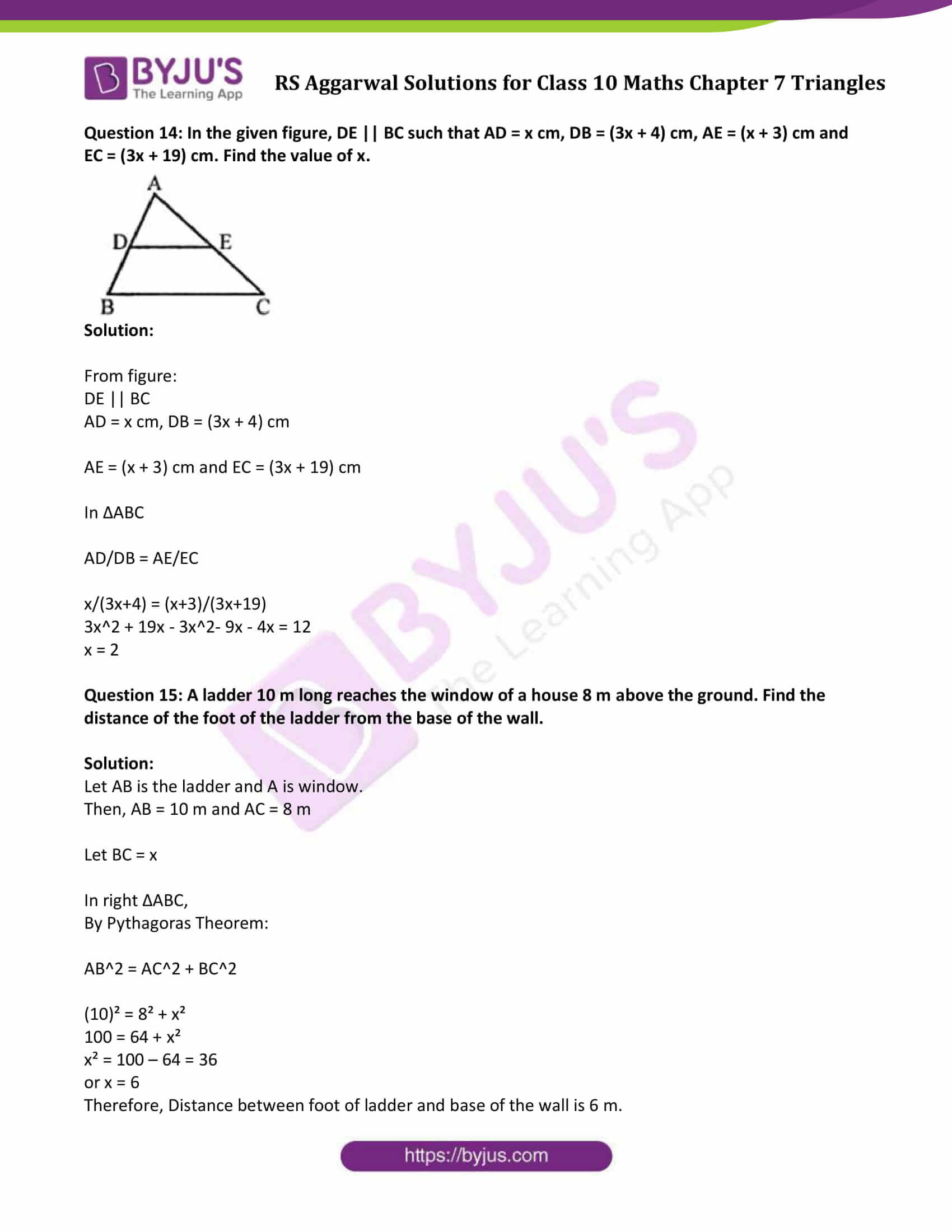### Access Answers to Maths R S Aggarwal Chapter 7 Triangles

#### Exercise 7A

Question 1: D and E are points on the sides AB and AC respectively of a ∆ABC such that DE || BC.

(i) If AD = 3.6 cm, AB = 10 cm and AE = 4.5 cm, find EC and AC.

(ii) If AB = 13.3 cm, AC = 11.9 cm and EC = 5.1 cm, find AD.

(iii) If AD/DB = 4/7 and AC = 6.6 cm, find AE.

(iv) if AD/AB = 8/15 and EC = 3.5 cm, find AE.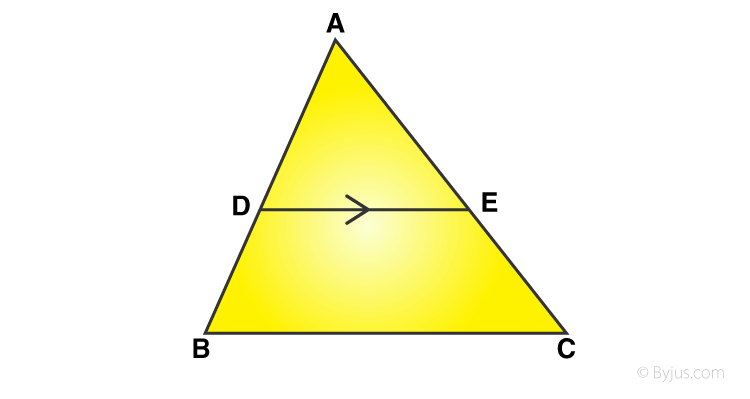Solution:

From given triangle, points D and E are on the sides AB and AC respectively such that DE || BC.

(i)AD = 3.6 cm, AB = 10 cm and AE = 4.5 cm.

By Thale’s Theorem:

Here DB = AB – AD = 10 – 3.6 = 6.4

=> EC = 4.6/3.6 x 6.4

or EC = 8

And, AC = AE + EC

AC = 4.5 + 8 = 12.5

(ii) If AB = 13.3 cm, AC = 11.9 cm and EC = 5.1 cm, find AD.

By Thale’s Theorem:

AD/DB + 1 = AE/EC + 1

(AD + DB)/DB = (AE + EC)/EC

AB/DB = AC/EC

or DB = (ABxEC)/ AC

= (13.3 x 5.1)/11.90

= 5.7

=> BD = 5.7

And, AD = AB – DB

(iii) AD/DB = 4/7 or AD = 4 cm, DB = 7 cm, and AC = 6.6

By Thale’s Theorem:

AD/DB + 1 = AE/EC + 1

(AD + DB)/DB = (AE + EC)/EC

(4+7)/7 = AC/EC = 6.6/EC

EC = (6.6 x 7)/11

= 4.2

And, AE = AC – EC

AE = 6.6 – 4.2

AE = 2.4 cm

(iv)

AD/AB = 8/15 or AD = 8 cm, AB = 15 cm, and EC = 3.5 cm

By Thale’s Theorem:

8/15 = AE/(AE+EC) = AE/(AE+3.5)

8(AE + 3.5) = 15AE

7 AE = 28

or AE = 4 cm

Question 2: D and E are points on the sides AB and AC respectively of a ∆ABC such that DE || BC. Find the value of x, when

(i) AD = x cm, DB = (x – 2) cm, AE = (x + 2) cm and EC = (x – 1) cm.

(ii) AD = 4 cm, DB = (x – 4) cm, AE = 8 cm and EC = (3x – 19) cm.

(iii) AD = (7x – 4) cm, AE = (5x – 2) cm, DB = (3x + 4) and EC = 3x cm.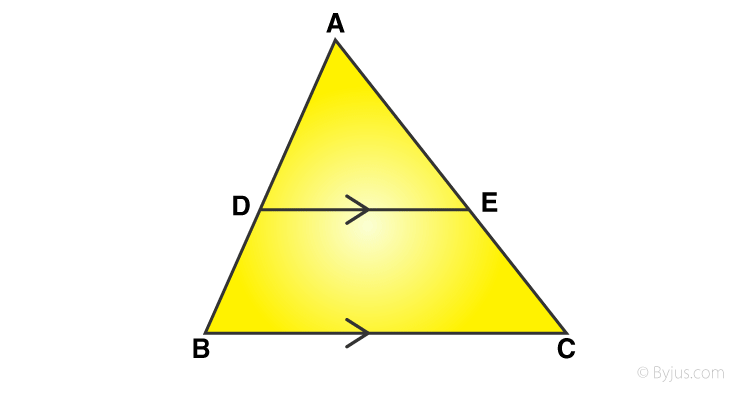Solution:

From figure, D and E are the points on the sides AB and AC respectively and DE || BC

(i) AD = x cm, DB = (x – 2) cm, AE = (x + 2) cm and EC = (x – 1) cm.

x/(x-2) = (x+2)/(x-1)

x(x-1) = (x+2)(x-2)

Solving above equation, we get

x = 4 cm

(ii) AD = 4 cm, DB = (x – 4) cm, AE = 8 cm and EC = (3x – 19) cm.

4/(x-4) = 8/(3x-19)

4(3x-19) = 8(x-4)

Solving, we get x = 11 cm

(iii)

AD = (7x – 4) cm, AE = (5x – 2) cm, DB = (3x + 4) and EC = 3x cm.

(7x-4)/(3x + 4) = (5x – 2)/3x

(7x – 4) (3x) = (5x – 2) (3x + 4)

21x² – 12x – 15x² – 20x + 6x = -8

6x² – 26x + 8 = 0

(x – 4) (3x – 1) = 0

Either x – 4 = 0 or (3x – 1) = 0

=> x = 4 or 1/3 (not possible)

So, x = 4

Question 3: D and E are points on the sides AB and AC respectively of a ∆ABC. In each of the following cases, determine whether DE || BC or not.

(i) AD = 5.7 cm, DB = 9.5 cm, AE = 4.8 cm and EC = 8 cm

(ii) AB = 11.7 cm, AC = 11.2 cm, BD = 6.5 cm and AE = 4.2 cm.

(iii) AB = 10.8 cm, AD = 6.3 cm, AC = 9.6 cm and EC = 4 cm.

(iv) AD = 7.2 cm, AE = 6.4 cm, AB = 12 cm and AC = 10 cm.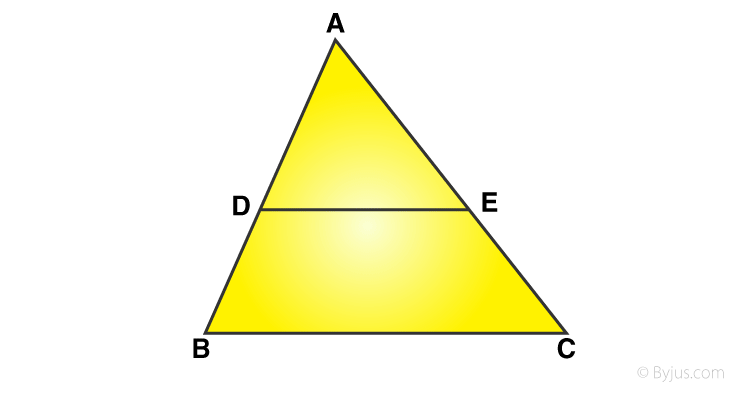Solution:

From figure, D and E are the points on the sides AB and AC of ∆ABC

(i) AD = 5.7 cm, DB = 9.5 cm, AE = 4.8 cm and EC = 8 cm

and AE/EC = 4.8/8 = 3/5

=> DE || BC

(ii) AB = 11.7 cm, AC = 11.2 cm, BD = 6.5 cm and AE = 4.2 cm.

AD = AB – BD = 11.7 – 6.5 = 5.2 cm and

EC = AC – AE = 11.2 – 4.2 = 7 cm

AE/EC = 4.2/7 = 3/5

=> DE is not parallel to BC

(iii) AB = 10.8 cm, AD = 6.3 cm, AC = 9.6 cm and EC = 4 cm.

DB = AB – AD = 10.8 – 6.3 = 4.5 cm

AE =AC – EC = 9.6 – 4 = 5.6 cm

AD /DB = 6.3/4.5 = 7/5

AE/EC = 5.6/4 = 7/5

=> DE || BC

(iv) AD = 7.2 cm, AE = 6.4 cm, AB = 12 cm and AC = 10 cm.

DB = AB – AD = 12 – 7.2 = 4.8 cm and

EC = AC – AE = 10 – 6,4 =3.6 cm

AD /DB = 7.2/4.8 = 3/2 and

AE/EC = 6.4/3.6 = 16/9

=> DE is not parallel to BC

Question 4: In a ∆ABC, AD is the bisector of ∠A.

(i) If AB = 6.4 cm, AC = 8 cm and BD = 5.6 cm, find DC.

(ii) If AB = 10 cm, AC = 14 cm and BC – 6 cm, find BD and DC.

(iii) If AB = 5.6 cm, BD = 3.2 cm and BC = 6 cm, find AC.

(iv) If AB = 5.6 cm, AC = 4 cm and DC = 3 cm, find BC.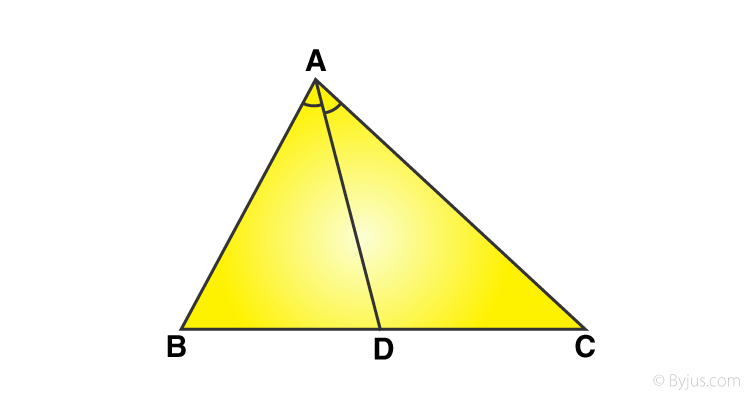Solution:

(i) If AB = 6.4 cm, AC = 8 cm and BD = 5.6 cm, find DC.

AD bisects ∠A, we can apply angle-bisector theorem in ∆ABC,

BD/DC = AB/AC

Substituting given values, we get

5.6/DC = 6.4/8

DC = 7 cm

(ii) If AB = 10 cm, AC = 14 cm and BC – 6 cm, find BD and DC.

By angle-bisector theorem

BD/DC = AB/AC = 10/14

Let BD = x cm and DC = (6-x) (As BC = 6 cm given)

x/(6-x) = 10/14

14x = 10(6 – x)

14x = 60 – 10x

14x + 10x = 60

or x = 2.5

Or BD = 2.5

Then DC = 6 – 2.5 = 3.5 cm

(iii) If AB = 5.6 cm, BD = 3.2 cm and BC = 6 cm, find AC.

BD/DC = AB/AC

Here DC = BC – BD = 6 – 3.2 = 2.8

=> DC = 2.8

3.2/2.8 = 5.6/AC

=> AC = 4.9 cm

(iv) If AB = 5.6 cm, AC = 4 cm and DC = 3 cm, find BC.

BD/DC = AB/AC

BD/3 = 5.6/4

=> BD = 4.2

Now, BC = BD + DC = 4.2 + 3 = 7.2

BC is 7.2 cm.

Question 5: M is a point on the side BC of a parallelogram ABCD. DM when produced meets AB produced at N. Prove that

(i) DM/MN = DC/BN (ii) DN/DM = AN/DC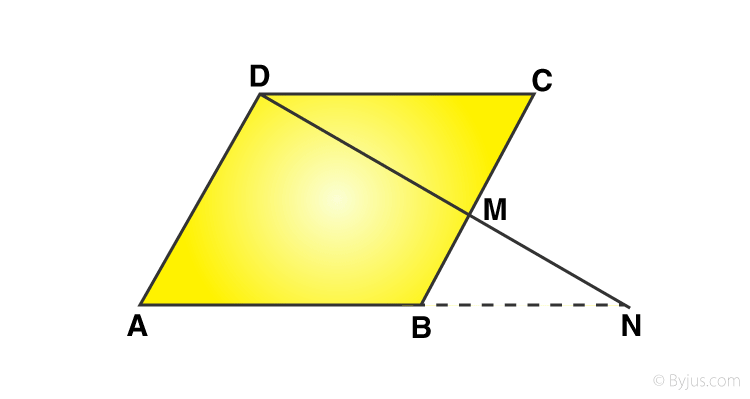Solution:

M is a point on the side BC of a parallelogram ABCD

(i)Consdier ∆DMC and ∆NMB,

 ∠DCM = ∠NBM alternate angles ∠DMC = ∠NMB vertically opposite angles ∠CDM = ∠MNB alternate angles

By By AAA-similarity:

∆DMC ∼ ∆NMB

From similarity of the triangle:

DM/MN = DC/BN

(ii)

From (i), DM/MN = DC/BN

DM/MN + 1 = DC/BN + 1

(DM+MN)/MN = (DC+BN)/BN

Since AB = CD

(DM+MN)/MN = (AB+BN)/BN

DN/DM = AN/DC

Hence proved.

Question 6: Show that the line segment which joins the midpoints of the oblique sides of a trapezium is parallel to the parallel sides.

Solution: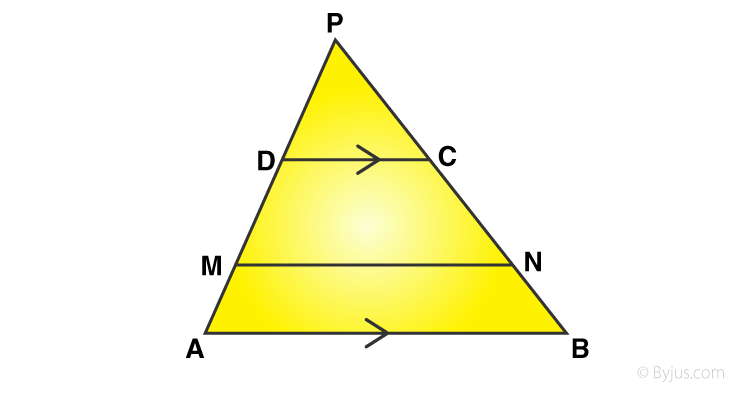Here, AB || DC

M and N are the mid points of sides AD and BC respectively.

MN is joined.

To prove : MN || AB or DC.

Produce AD and BC to meet at P.

Now, In ∆PAB

DC ||AB

PD/DA = PC/CB

PD/2PM = PC/2CN

M and N are midpoints of AD and BC respectively.

PD/PM = PC/CN

MN||DC But DC ||AB

Therefore, MN || DC ||AB

Question 7: In the adjoining figure, ABCD is a trapezium in which CD || AB and its diagonals intersect at O. If AO = (5x – 7) cm, OC = (2x + 1) cm, BO = (7x – 5) cm and OD = (7x + 1) cm, find the value of x.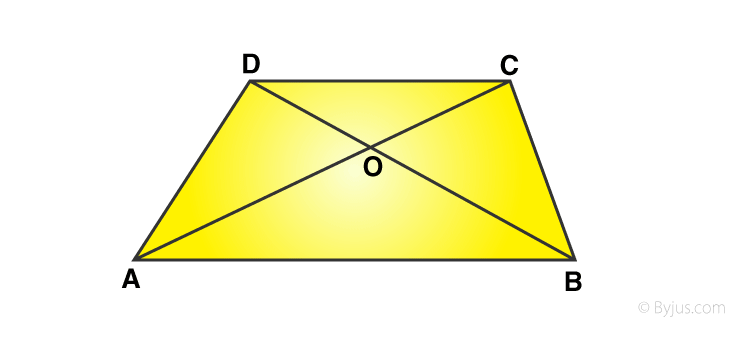Solution:

From given statement:

EO || AB || DC

By thales theorem: AE/ED = AO/OC …(1)

In Δ DAB,

EO || AB

So, By thales theorem: DE/EA = DO/OB …(2)

From (1) and (2)

AO/OC = DO/OB

(5x – 7) / (2x + 1) = (7x-5) / (7x+1)

(5x – 7)(7x + 1) = (7x – 5)(2x + 1)

35x^2 + 5x – 49x – 7 = 14x^2 – 10x + 7x – 5

35x^2 – 14x^2 – 44x + 3x – 7 + 5 = 0

21x^2 – 42x + x – 2 = 0

21(x – 2) + (x – 2) = 0

(21x + 1)(x – 2) = 0

Either (21x + 1) = 0 or (x – 2) = 0

x = -1/21 (does not satisfy) or x = 2

=> x = 2.

Question 8: In a ∆ABC, M and N are points on the sides AB and AC respectively such that BM = CN. If ∠B = ∠C then show that MN || BC.

Solution:

In ∆ABC, M and N are points on the sides AB and AC respectively such that BM = CN and if ∠B = ∠C.

We know that, sides opposite to equal angles are equal.

AB = AC

BM = CN ( given)

AB – BM = AC – CN

=> AM = An

From ∆ABC

AM/MB = AN/NC

Therefore, MN ||BN

#### Exercise 7B

Question 1: In each of the given pairs of triangles, find which pair of triangles are similar. State the similarity criterion and write the similarity relation in symbolic form: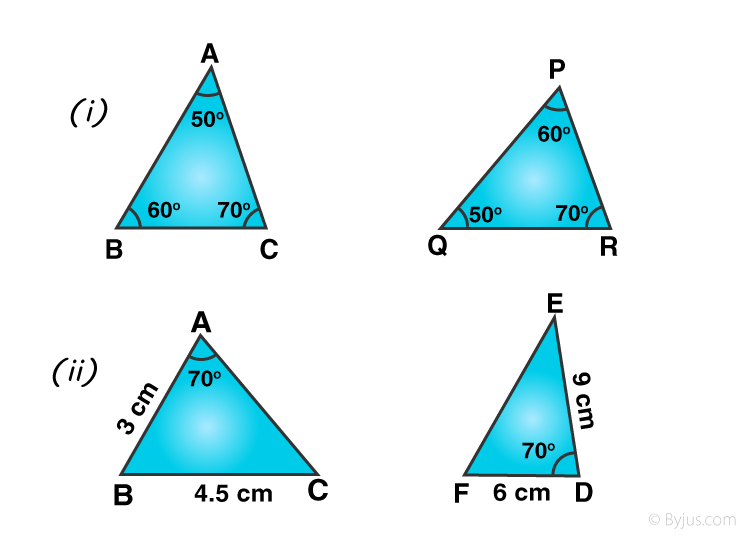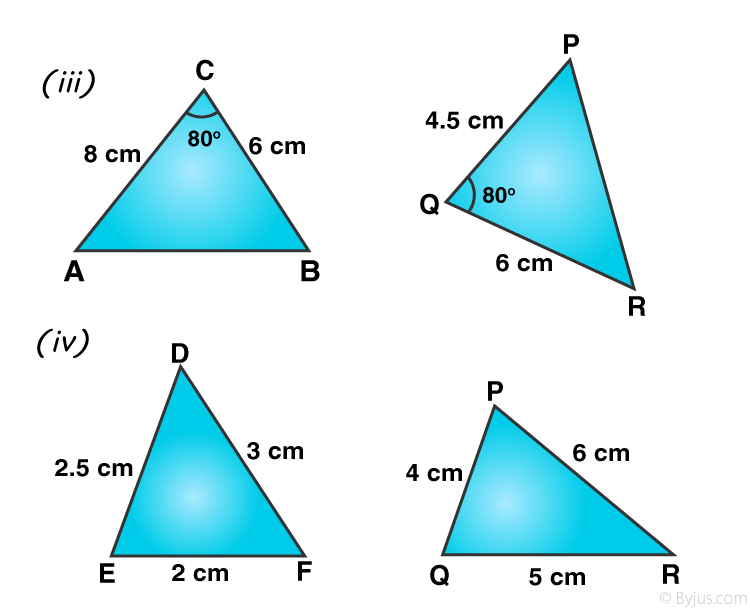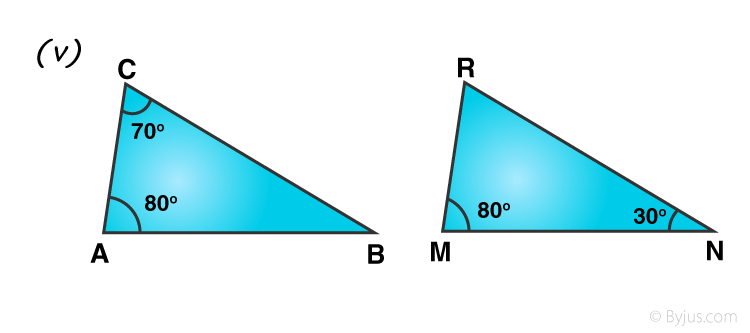Solution:

Two triangles are similarity of their corresponding angles are equal and corresponding sides are proportional.

(i) In ∆ABC and ∆PQR

∠A = ∠Q = 50°

∠B = ∠P = 60° and

∠C = ∠R = 70°

∆ABC ~ ∆QPR (By AAA)

(ii) In ∆ABC and ∆DEF

In ∆ABC and ∆DEF

AB = 3 cm, BC = 4.5

DF = 6 cm, DE = 9 cm

∆ABC is not similar to ∆DEF

(iii) In ∆ABC and ∆PQR

In ∆ABC and ∆PQR

AC = 8 cm BC = 6 cm

Included ∠C = 80°

PQ = 4.5 cm, QR = 6 cm

and included ∠Q = 80°

AC/QR = 8/6 = 4/3

and BC/PQ = 6/4.5 = 4/3

=> AC/QR = BC/PQ

and ∠C = ∠Q = 80°

∆ABC ~ ∆PQR (By SAS)

(iv)In ∆DEF and ∆PQR

DE = 2.5, DF = 3 and EF = 2

PQ = 4, PR = 6 and QR = 5

DE/QR = 2.5/5 = 1/2

DF/PR = 3/6 = 1/2

and EF/PQ = 2/4 = 1/2

∆DEF ~ ∆PQR (By SSS)

(v) In ∆ABC and ∆MNR

∠A = 80°, ∠C = 70°

So, ∠B = 180° – (80° + 70°) = 30°

∠M = 80°, ∠N = 30°, and ∠R = 180° – (80° + 30°) = 70°

Now, in ∆ABC

∠A = ∠M – 80°, ∠B = ∠N = 30°

and ∠C = ∠R = 70°

∆ABC ~ ∆MNR (By AAA or AA)

Question 2: In the given figure, ∆ODC ~ ∆OBA, ∠BOC = 115° and ∠CDO = 70°. Find:

(i) ∠DOC

(ii) ∠DCO

(iii) ∠OAB

(iv) ∠OBA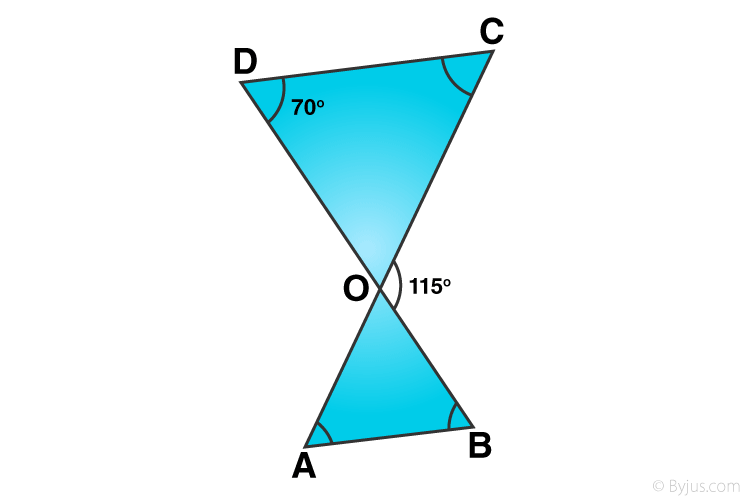Solution:

Here ∆ODC ~ ∆OBA, so

∠D = ∠B = 70°

∠C = ∠A

∠COD = ∠AOB

(i) But ∠DOC + ∠BOC = 180° (Linear pair)

∠DOC + 115°= 180°

∠DOC = 180° – 115° = 65°

(ii) ∠DOC + ∠CDO + ∠DCO = 180° (Angles of a triangle)

65° + 70° + ∠DCO = 180°

135° + ∠DCO = 180°

∠DCO = 180° – 135° = 45°

(iii) ∠AOB = ∠DOC = 65° (vertically opposite angles)

∠OAB = ∠DCO = 45° (Since ∆ODC ~ ∆OBA)

(iv) ∠OBA = ∠CDO = 70° (Since ∆ODC ~ ∆OBA)

Question 3: In the given figure, ∆OAB ~ ∆OCD. If AB = 8 cm, BO = 6.4 cm, OC = 3.5 cm and CD = 5 cm, find (i) OA (ii) DO.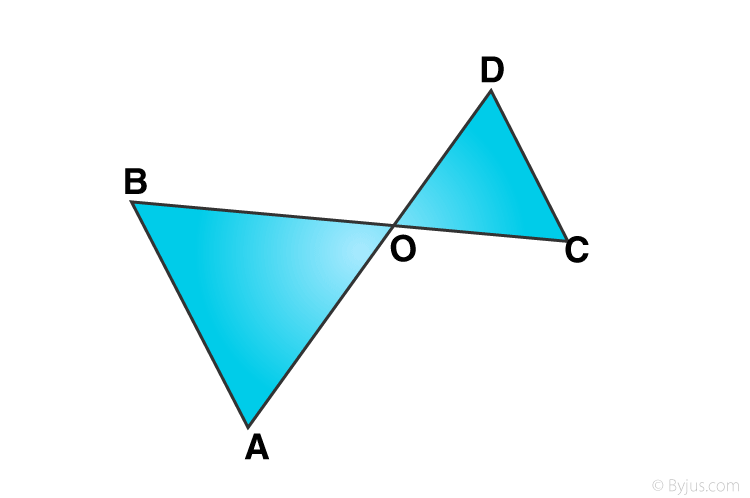Solution:

Since ∆OAB ~ ∆OCD

AB = 8 cm, BO = 6.4 cm OC = 3.5 cm, CD = 5 cm

Let OD = y and OA = x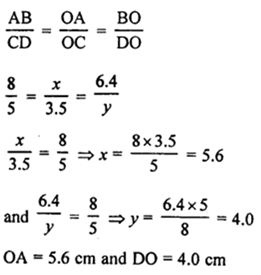Question 4: In the given figure, if ∠ADE = ∠B, show that ∆ADE ~ ∆ABC. If AD = 3.8 cm, AE = 3.6 cm, BE = 2.1 cm and BC = 4.2 cm, find DE.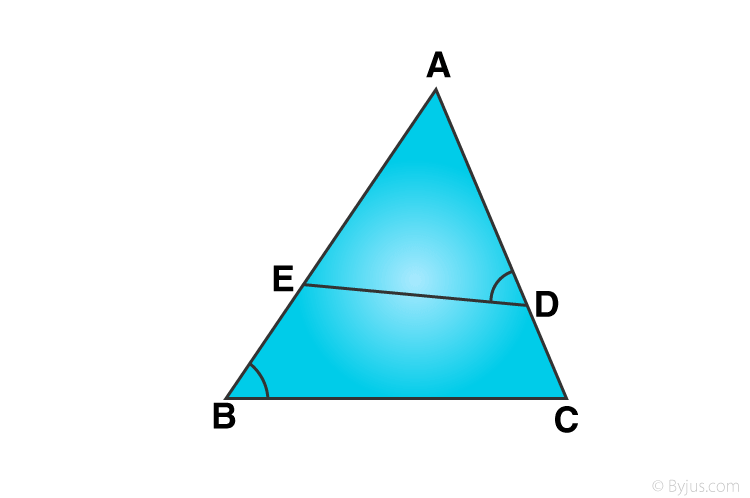Solution:

From given figure,

To prove:

find DE

Given: AD = 3.8 cm, AE = 3.6 cm, BE = 2.1 cm and BC = 4.2 cm

∠A = ∠A (common)

Again,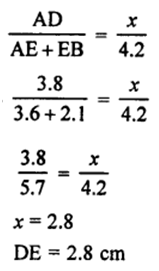Question 5: The perimeters of two similar triangles ABC and PQR are 32 cm and 24 cm respectively. If PQ = 12 cm, find AB.

Solution:

Form given statement: ∆ABC ~ ∆PQR,

PQ = 12 cm

Perimeter of ∆ABC = AB + BC + CA = 32 cm

Perimeter of ∆PQR = PQ + QR + RP = 24 cm

Now,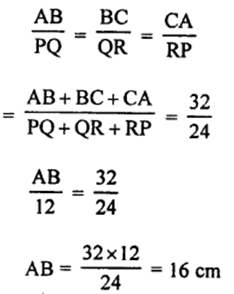Question 6: The corresponding sides of two similar triangles ABC and DEF are BC = 9.1 cm and EF = 6.5 cm. If the perimeter of ∆DEF is 25 cm, find the perimeter of ∆ABC.

Solution:

Form given statement: ∆ABC ~ ∆DEF

BC = 9.1 cm, EF = 6.5 cm and Perimeter of ∆DEF = 25 cm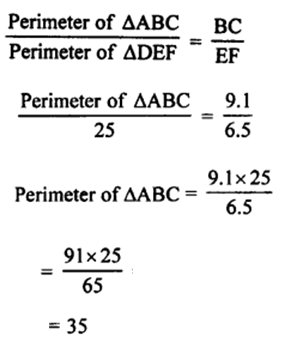Perimeter of ∆ABC is 35 cm

Question 7: In the given figure, ∠CAB = 90° and AD ⊥ BC. Show that ∆BDA ~ ∆BAC. If AC = 75 cm, AB = 1 m and BC = 1.25 m, find AD.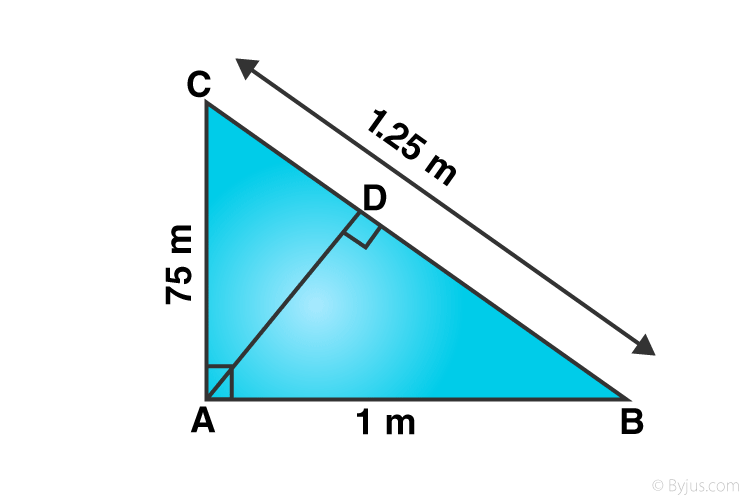Solution:

∠CAB = 90° and AD ⊥ BC

If AC = 75 cm, AB = 1 m or 100 cm, BC = 1.25 m or 125 cm

∠BDA = ∠BAC = 90°

∠DBA = ∠CBA [common angles]

By AA

∆BDA ~ ∆BAC

And,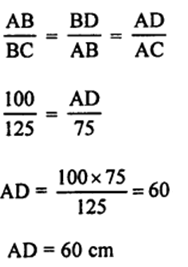Question 8: In the given figure, ∠ABC = 90° and BD ⊥ AC. If AB = 5.7 cm, BD = 3.8 cm and CD = 5.4 cm, find BC.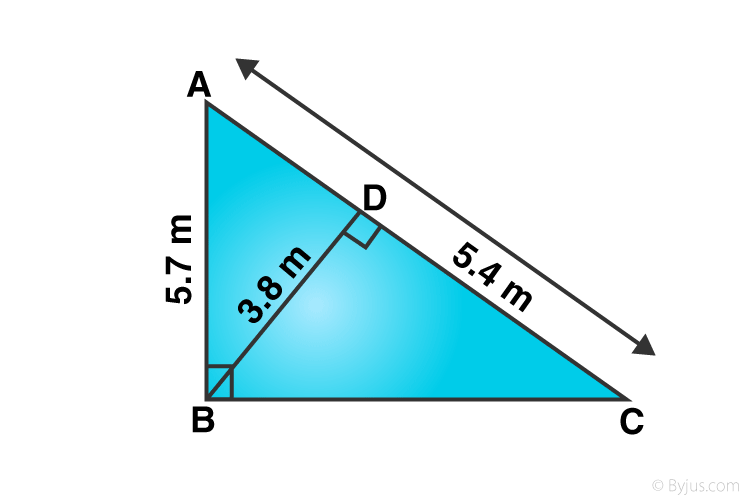Solution:

From given:

∠ABC = 90°, BD ⊥ AC.

AB = 5.7 cm, BD = 3.8 cm, CD = 5.4 cm

In ∆ABC and ∆BDC,

∠ABC = ∠BDC (each 90°)

∠BCA = ∠BCD (common angles)

∆ABC ~ ∆BDC (AA axiom)

So, corresponding sides are proportional

AB/BD = BC/CD

=> 5.7/3.8 = BC/5.4

=> BC = 8.1

#### Exercise 7C

Question 1: ∆ABC ~ ∆DEF and their areas are respectively 64 cm² and 121 cm². If EF = 15.4 cm, find BC.

Solution:

Area of ∆ABC = 64 cm² and

area of ∆DEF = 121 cm²

EF = 15.4 cm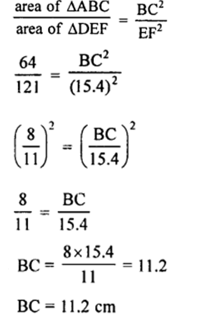Question 2: The areas of two similar triangles ABC and PQR are in the ratio 9 : 16. If BC = 4.5 cm, find the length of QR.

Solution:

The areas of two similar triangles ABC and PQR are in the ratio 9 : 16.

BC = 4.5 cm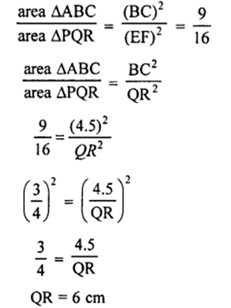Question 3: ∆ABC ~ ∆PQR and ar (∆ABC) = 4ar (∆PQR). If BC = 12 cm, find QR.

Solution:

∆ABC ~ ∆PQR

ar (∆ABC) = 4ar (∆PQR)Question 4: The areas of two similar triangles are 169 cm² and 121 cm² respectively. If the longest side of the larger triangle is 26 cm, find the longest side of the smaller triangle.

Solution:

Areas of two similar triangles are 169 cm² and 121 cm² (given)

Longest side of largest triangle = 26 cm

Let longest side of smallest triangle is x cm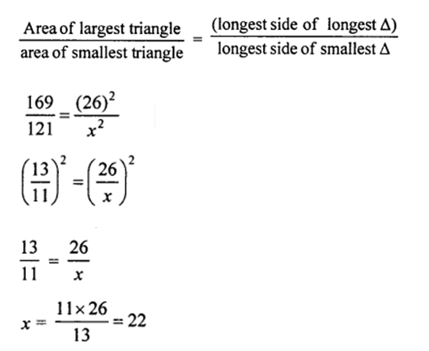Longest side of smallest triangle is 22 cm

Question 5: ∆ABC ~ ∆DEF and their areas are respectively 100 cm² and 49 cm². If the altitude of ∆ABC is 5 cm, find the corresponding altitude of ∆DEF.

Solution:

Area of ∆ABC = 100 cm²

area of ∆DEF = 49 cm²

Altitude of ∆ABC is 5 cm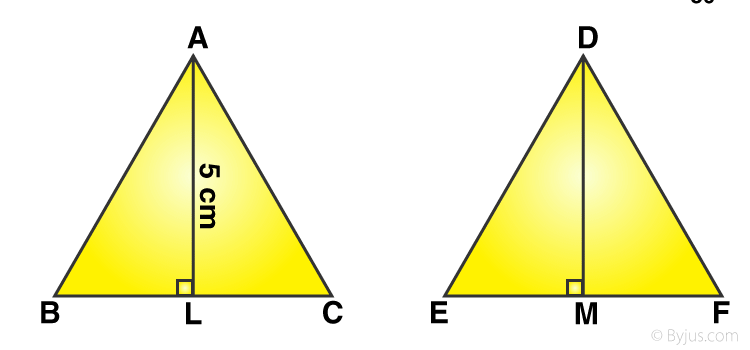AL ⊥ BC and DM ⊥ EF

Let DM = x cm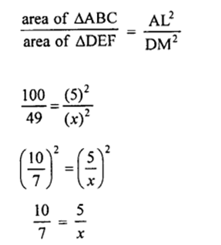Or x = 3.5

Altitude of smaller triangle is 3.5 cm

Question 6: The corresponding altitudes of two similar triangles are 6 cm and 9 cm respectively. Find the ratio of their areas.

Solution:

Corresponding altitudes of two similar triangles are 6 cm and 9 cm (given)

We know that the areas of two similar triangles are in the ratio of the squares of their corresponding altitudes.

Ratio in the areas of two similar triangles = (6)² : (9)² = 36 : 81 = 4 : 9

Question 7: The areas of two similar triangles are 81 cm² and 49 cm² respectively. If the altitude of the first triangle is 6.3 cm, find the corresponding altitude of the other.

Solution:

Areas of two similar triangles are 81 cm² and 49 cm²

Altitude of the first triangle = 6.3 cm

Let altitude of second triangle = x cm

Area of ∆ABC = 81 cm² and area of ∆DEF = 49cm²

Altitude AL = 6 – 3 cm

Let altitude DM = x cm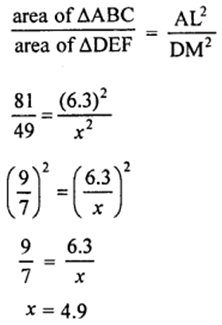Altitude of second triangle is 4.9 cm

Question 8: The areas of two similar triangles are 100 cm² and 64 cm² respectively. If a median of the smaller triangle is 5.6 cm, find the corresponding median of the other.

Solution:

Areas of two similar triangles are 100 cm² and 64 cm²

Median DM of ∆DEF = 5.6 cm

Let median AL of ∆ABC = x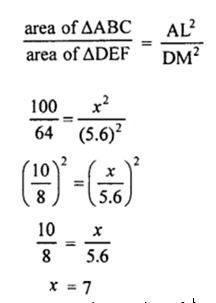Corresponding median of the other triangle is 7 cm.

Question 9: In the given figure, ABC is a triangle and PQ is a straight line meeting AB in P and AC in Q. If AP = 1 cm, PB = 3cm, AQ = 1.5 cm, QC = 4.5 cm, prove that area of ∆APQ is 1/16 of the area of ∆ABC.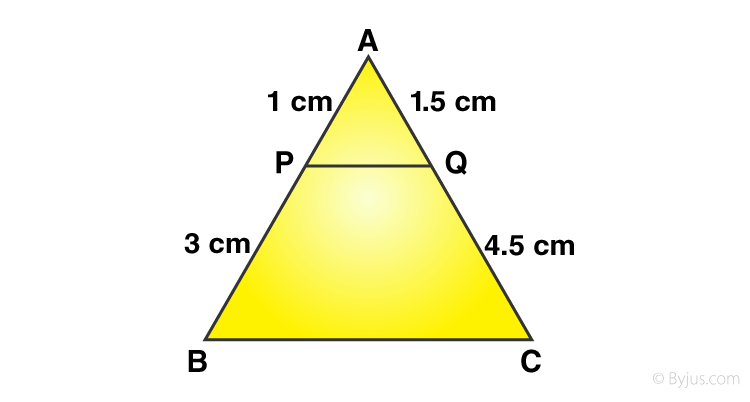Solution:

In ∆ABC, PQ is a line which meets AB in P and AC in Q.

Given: AP = 1 cm, PB = 3 cm, AQ = 1.5 cm QC = 4.5 cm

Now, AP/PB = 1/3 and AQ/QC = 1.5/4.5 = 1/3

=> AP/PB = AQ/QC

From figure: AB = AP + PB = 1+3 = 4 cm

AC = AQ + QC = 1.5 + 4.5 = 6 cm

In ∆APQ and ∆ABC,

AP/AB = AQ/AC

angle A (common)

∆APQ and ∆ABC are similar triangles.

Now,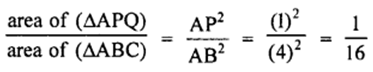Which implies,

area of ∆APQ = 1/16 of the area of ∆ABC

Hence Proved.

Question 10: In the given figure, DE || BC. If DE = 3 cm, BC = 6 cm and ar (∆ADE) = 15 cm², find the area of ∆ABC.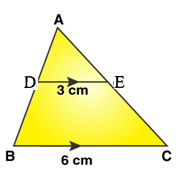Solution:

DE || BC

DE = 3 cm, BC = 6 cm

Now,

In ∆ABC

DE ||BC. Therefore triangles, ∆ADE and ∆ABC are similar.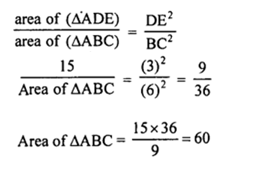Area of ∆ABC is 60 cm2.

#### Exercise 7D

Question 1: The sides of certain triangles are given below. Determine which of them are right triangles.

(i) 9 cm, 16 cm, 18 cm

(ii) 1 cm, 24 cm, 25 cm

(iii) 1.4 cm, 4.8 cm, 5 cm

(iv) 1.6 cm, 3.8 cm, 4 cm

(v) (a – 1) cm, 2√a cm, (a + 1) cm

Solution:

A given triangle to be right-angled, if it satisfies Pythagorean Theorem. That is, the sum of the squares of the two smaller sides must be equal to the square of the largest side.

(i) 9 cm, 16 cm, 18 cm

Longest side = 18

Now (18)² = 324

and (9)² + (16)² = 81 + 256 = 337

324 ≠ 337

It is not a right triangle.

(ii) 1 cm, 24 cm, 25 cm

Longest side = 25 cm

(25)² = 625

and (7)² x (24)² = 49 + 576 = 625

625 = 625

It is a right triangle.

(iii) 1.4 cm, 4.8 cm, 5 cm

Longest side = 5 cm

(5)² = 25

and (1.4)² + (4.8)² = 1.96 + 23.04 = 25.00 = 25

25 = 25

It is a right triangle.

(iv) 1.6 cm, 3.8 cm, 4 cm

Longest side = 4 cm

(4 )² = 16

and (1.6)² + (3.8)² = 2.56 + 14.44 = 17.00 = 17

16 ≠ 17

It is not a right triangle.

(v) (a- 1) cm, 2√a cm, (a + 1) cm

Longest side = (a + 1) cm

(a + 1)² = a² + 2a + 1

and (a – 1)² + (2 √a )² = a² – 2a + 1 + 4a = a² + 2a + 1

a² + 2a + 1 = a² + 2a + 1

It is a right triangle.

Question 2: A man goes 80 m due east and then 150 m due north. How far is he from the starting point?

Solution:

A man goes 80 m from O to east side and reaches A, then he goes 150 m due north from A and reaches B.

Draw a figure based on given instructions: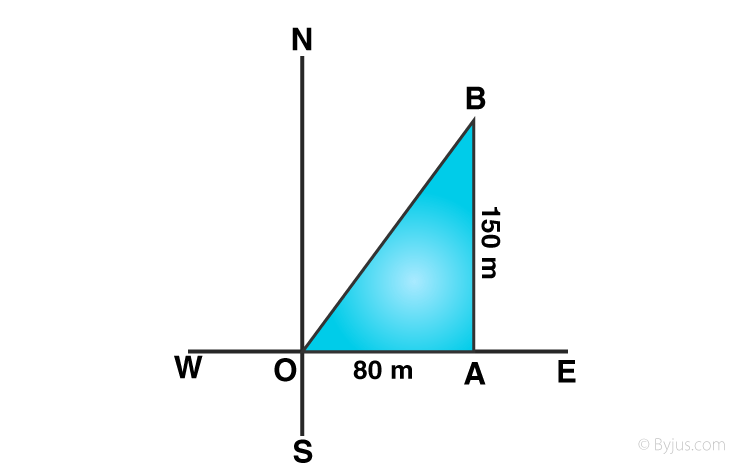From right ∆OAB,

By Pythagoras Theorem:

OB2 = OA2+ AB2

= (80)² + (150)²

= 6400 + 22500

= 28900

or OB = √28900 = 170

Man is 170 m away from the starting point.

Question 3: A man goes 10 m due south and then 24 m due west. How far is he from the starting point?

Solution:

A man goes 10 m due south from O and reaches A and then 24 m due west from A and reaches B.

Draw a figure based on given instructions: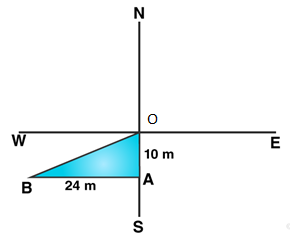From right ∆OAB,

By Pythagoras Theorem:

OB2 = OA2+ AB2

= (10)² + (24)²

= 676

or OB = 26

Man is 26 m away from the starting point.

Question 4: A 13-m-long ladder reaches a window of a building 12 m above the ground. Determine the distance of the foot of the ladder from the building.

Solution:

Height of the window = 12 m

Length of a ladder = 13 m

In the figures,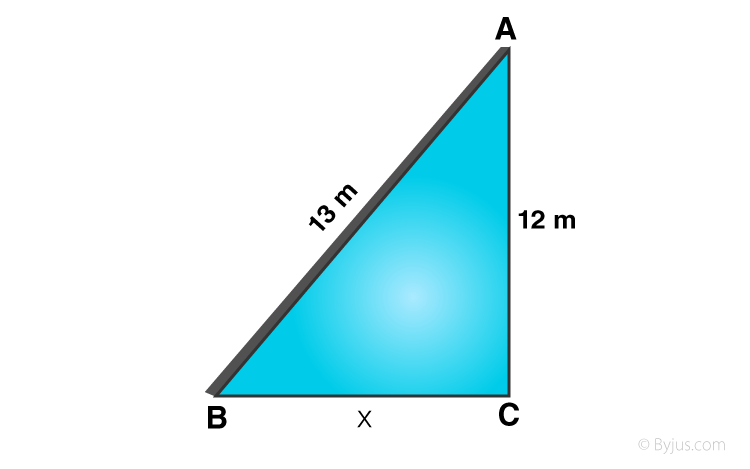Let AB is ladder, A is window of building AC

By Pythagoras Theorem:

AB2 = AC2 + BC2

(13)² = (12)² + x²

169 = 144 + x²

x² = 169 – 144 = 25

or x = 5

Distance between foot of ladder and building = 5 m.

Question 5: A ladder is placed in such a way that its foot is at a distance of 15 m from a wall and its top reaches a window 20 m above the ground. Find the length of the ladder.

Solution:

Height of window AC = 20 m

Let length of ladder AB = x m

Distance between the foot of the ladder and the building (BC) = 15 m

In the figure: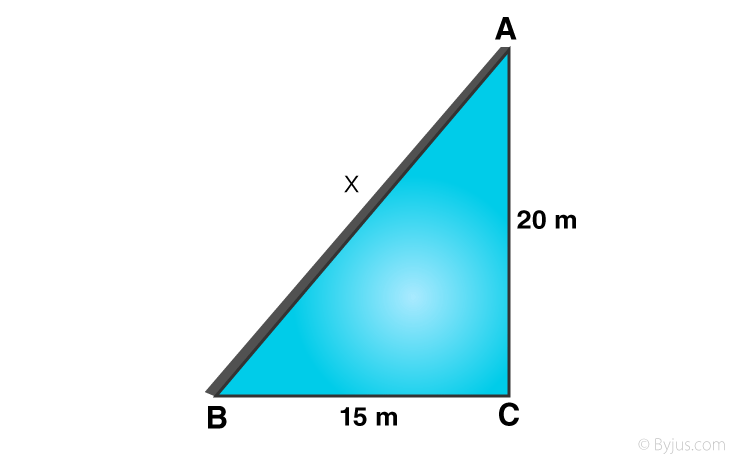By Pythagoras Theorem:

AB2 = AC2 + BC2

x² = 20² + 15²

= 400 + 225

= 625

or x = 25

Length of ladder is 25 m

Question 6: Two vertical poles of height 9 m and 14 m stand on a plane ground. If the distance between their feet is 12 m, find the distance between their tops.

Solution:

Height of first pole AB = 9 m and

Height of second pole CD = 14 m

Let distance between their tops CA = x m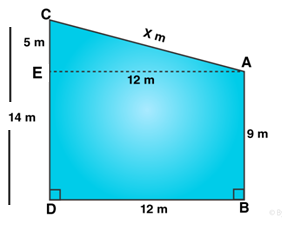From A, draw AE || BD meeting CD at E.

Then EA = DB = 12 m CE = CD – ED = CD – AB = 14-9 = 5 m

In right ∆AEC,

AC² = AE² + CE²

= 122 + 52

= 144 + 25

= 169

or AC = 13

Distance between pole’s tops is 13 m

Question 7: A guy wire attached to a vertical pole of height 18 m is 24 m long and has a stake attached to the other end. How far from the base of the pole should the stake be driven so that the wire will be taut?

Solution:

Length of wire = AC = 24 m

Height of the pole = AB = 18 m

Let Distance between the base of the pole and other end of the wire = BC = x m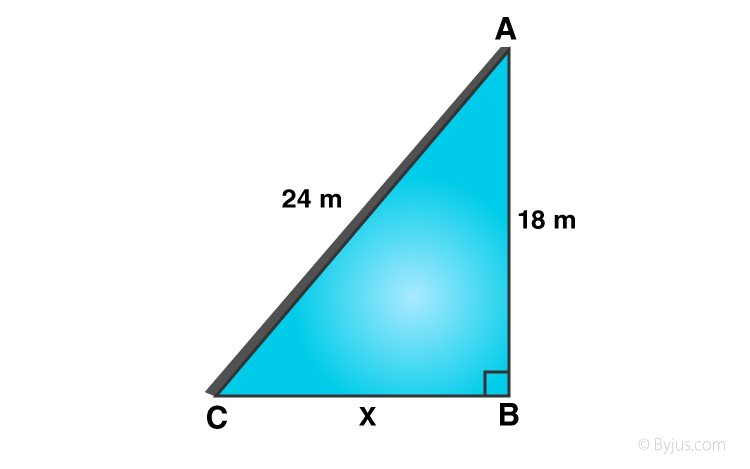In right ∆ABC,

By Pythagoras Theorem:

AC2 = AB2 + BC2

(24)² = (18)² + x²

576 = 324 + x²

x² = 576 – 324 = 252

or x = 6v7

BC is 6v7m

Question 8: In the given figure, O is a point inside a ∆PQR such that ∠POR = 90°, OP = 6 cm and OR = 8 cm. If PQ = 24 cm and QR = 26 cm, prove that ∆PQR is right-angled.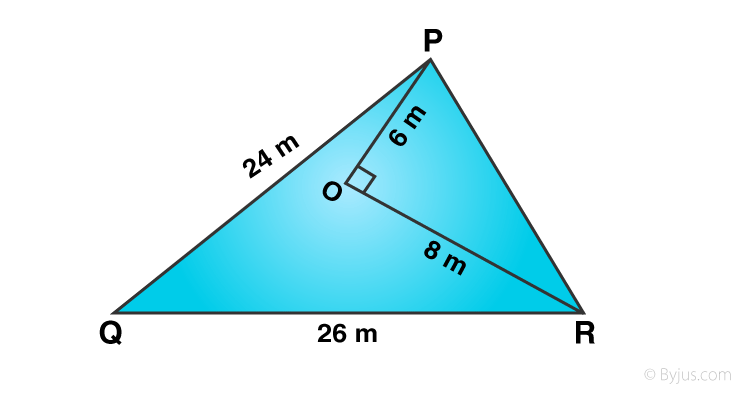Solution:

In ∆PQR, O is a point in it such that

OP = 6 cm, OR = 8 cm and ∠POR = 90°

PQ = 24 cm, QR = 26 cm

Now,

In ∆POR, ∠O = 90°

PR² = PO² + OR²

= (6)² + (8)²

= 36 + 64

= 100

PR = 10

Greatest side QR is 26 cm

QR² = (26)² = 676

and PQ² + PR² = (24)² + (10)²

= 576 + 100

= 676

Which implies, 676 = 676

QR² = PQ² + PR²

∆PQR is a right angled triangle and right angle at P.

Question 9: ∆ABC is an isosceles triangle with AB = AC = 13 cm. The length of altitude from A on BC is 5 cm. Find BC.

Solution:

In isosceles ∆ABC, AB = AC = 13 cm

Consider AL is altitude from A to BC and AL = 5 cm

Now, in right ∆ALB

AB2 = AL2 + BL2

(13)² = (5)² + BL²

169 = 25 + BL²

BL² = 169 – 25 = 144

or BL = 12

Since L is midpoint of BC, then

BC = 2 x BC = 2 x 12 = 24

BC is 24 cm

Question 10: Find the length of altitude AD of an isosceles ∆ABC in which AB = AC = 2a units and BC = a units.

Solution:

In an isosceles ∆ABC in which AB = AC = 2a units, BC = a units

AD is the altitude. Therefore, D is the midpoint of BC

=> BD = a/2

By Pythagoras theorem,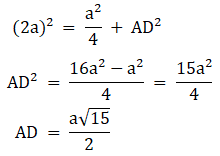#### Exercise 7E

Question 1: State the two properties which are necessary for given two triangles to be similar.

Solution:

Two properties for similarity of two triangles are:

(i) Angle-Angle-Angle (AAA) property.

(ii) Angle-Side-Angle (ASA) property.

Question 2: State the basic proportionality theorem.

Solution:

In a triangle, if a line parallel to one side is drawn, it will divide the other two sides proportionally.

Question 3: State the converse of Thales’ theorem.

Solution:

If a line divides any two sides of a triangle in the same ratio. Then, the line must be parallel to the third side.

Question 4: State the midpoint theorem.

Solution:

The line joining the midpoints of two sides of a triangle, is parallel to the third side.

Question 5: State the AAA-similarity criterion.

Solution:

In two triangles, if three angles of the one triangle are equal to the three angles of the other, the triangles are similar.

Question 6: State the AA-similarity criterion.

Solution:

In two triangles, if two angles of the one triangle are equal to the corresponding angles of the other triangle, then the triangles are similar.

Question 7: State the SSS-criterion for similarity of triangles.

Solution:

In two triangles, if three sides of the one are proportional to the corresponding sides of the other, the triangles are similar.

Question 8: State the SAS-similarity criterion.

Solution:

In two triangles, if two sides of the one are proportional to the corresponding sides of the other and their included angles are equal, the two triangles are similar.

Question 9: State Pythagoras’ theorem.

Solution:

In a right angled triangle, the square on the hypotenuse is equal to the sum of squares on the other two sides.

Question 10: State the converse of Pythagoras theorem.

Solution:

In a triangle, if the square on the longest side is equal to the sum of the squares on the other two sides, then the angle opposite to the hypotenuse is a right angle.

Question 11: If D, E and F are respectively the midpoints of sides AB, BC and CA of ∆ABC then what is the ratio of the areas of ∆DEF and ∆ABC?

Solution:

The ratio of their areas will be 1 : 4.

Question 12: Two triangles ABC and PQR are such that AB = 3 cm, AC = 6 cm, ∠A = 70°, PR = 9 cm, ∠P = 70° and PQ = 4.5 cm. Show that ∆ABC ~ ∆PQR and state the similarity criterion.

Solution:

In two triangles ∆ABC and ∆PQR,

AB = 3 cm, AC = 6 cm, ∠A = 70°

PR = 9 cm, ∠P = 70° and PQ= 4.5 cm

Now,

∠A = ∠P = 70° (Same)

AC/PR = 6/9 = 2/3 and

AB/PQ = 3/4.5 = 2/3

=> AC/PR = AB/PQ

Both ∆ABC and ∆PQR are similar.

Question 13: If ∆ABC ~ ∆DEF such that 2AB = DE and BC = 6 cm, find EF.

Solution:

∆ABC ~ ∆DEF (given)

2AB = DE, BC = 6 cm (given)

∠E = ∠B and ∠D = ∠A and ∠F = ∠C

2AB = DE

=> AB/DE = 1/2

Therefore,

AB/DE = BC/EF

1/2 = 6/EF

or EF = 12 cm

Question 14: In the given figure, DE || BC such that AD = x cm, DB = (3x + 4) cm, AE = (x + 3) cm and EC = (3x + 19) cm. Find the value of x.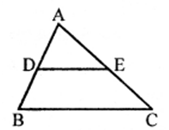Solution:

From figure:

DE || BC

AD = x cm, DB = (3x + 4) cm

AE = (x + 3) cm and EC = (3x + 19) cm

In ∆ABC

x/(3x+4) = (x+3)/(3x+19)

3x2 + 19x – 3x2– 9x – 4x = 12

x = 2

Question 15: A ladder 10 m long reaches the window of a house 8 m above the ground. Find the distance of the foot of the ladder from the base of the wall.

Solution:

Let AB is the ladder and A is window.

Then, AB = 10 m and AC = 8 m

Let BC = x

In right ∆ABC,

By Pythagoras Theorem:

AB2 = AC2 + BC2

(10)² = 8² + x²

100 = 64 + x²

x² = 100 – 64 = 36

or x = 6

Therefore, Distance between foot of ladder and base of the wall is 6 m.

## R S Aggarwal Solutions For Class 10 Maths Chapter 7 Exercises:

Get detailed solutions for all the questions listed under the below exercises:

Exercise 7 A Solutions

Exercise 7 B Solutions

Exercise 7 C Solutions

Exercise 7 D Solutions

Exercise 7 E Solutions

## R S Aggarwal Solutions for Class 10 Maths Chapter 7 Triangles

In this chapter students will study important concepts of triangles as listed below:

• Triangles introduction
• Congruent figures
• Similar figures
• Similar Polygons
• Equiangular triangles
• Similar Triangles
• Important Theorems on Triangles:
• Thales’ Theorem and it’s converse
• Midpoint theorem
• Angle-Bisector Theorem
• Criteria for similarity of two triangles
• Ratio of the Areas of two similar triangles
• Pythagoras Theorem
• Similar Triangles
• Thales’ Theorem and it’s converse
• Midpoint theorem

### Key Features of R S Aggarwal Solutions for Class 10 Maths Chapter 7 Triangles

1. Students will learn how to solve problems on triangles with the help of various solved problems.

2. Easy and simple language is used.

3. All questions are solved using step-by-step problem solving approach.